## 4. Content Markup

### 4.1. Introduction

#### 4.1.1. The Intent of Content Markup

As has been noted in the introductory section of this recommendation, mathematics can be distinguished by its use of a (relatively) formal language, mathematical notation. However, mathematics and its presentation should not be viewed as one and the same thing. Mathematical sums or products exist and are meaningful to many applications completely without regard to how they are rendered aurally or visually. The intent of the content markup in Mathematical Markup Language is to provide an explicit encoding of the underlying mathematical structure of an expression, rather than any particular rendering for the expression.

There are many reasons for providing a specific encoding for content. Even a disciplined and systematic use of presentation tags cannot properly capture this semantic information. This is because without additional information it is impossible to decide if a particular presentation was chosen deliberately to encode the mathematical structure or simply to achieve a particular visual or aural effect. Furthermore, an author using the same encoding to deal with both the presentation and mathematical structure might find a particular presentation encoding unavailable simply because convention had reserved it for a different semantic meaning.

The difficulties stem from the fact that there are many to one mappings from presentation to semantics and vice versa. For example the mathematical construct H multiplied by e' is often encoded using an explicit operator as in H × e. In different presentational contexts, the multiplication operator might be invisible H e', or rendered as the spoken word times'. Generally, many different presentations are possible depending on the context and style preferences of the author or reader. Thus, given H e' out of context it may be impossible to decide if this is the name of a chemical or a mathematical product of two variables H and e.

Mathematical presentation also changes with culture and time: some expressions in combinatorial mathematics today have one meaning to an English mathematician, and quite another to a French mathematician. Notations may lose currency, for example the use of musical sharp and flat symbols to denote maxima and minima. [Chaudry1954] A notation in use in 1644 for the multiplication mentioned above wasHe [Cajori1928].

When we encode the underlying mathematical structure explicitly, without regard to how it is presented aurally or visually, we are able to interchange information more precisely with those systems which are able to manipulate the mathematics. In the trivial example above, such a system could substitute values for the variables H and e and evaluate the result. Further interesting application areas include interactive textbooks and other teaching aids.

#### 4.1.2. The Scope of Content Markup

The semantics of general mathematical notation is not a matter of consensus. It would be an enormous job to systematically codify most of mathematics - a task which can never be complete. Instead, MathML makes explicit a relatively small number of commonplace mathematical constructs, chosen carefully to be sufficient in a large number of applications. In addition, it provides a mechanism for associating semantics with new notational constructs. In this way, mathematical concepts that are not in the base collection of tags can still be encoded (section 4.2.7 [Syntax and Semantics]).

The base set of content elements are chosen to be adequate for simple coding of most of the formulas used from kindergarten to the end of high school in the United States, and probably beyond through the first two years of college, that is up to A-Level or Baccalaureate level in Europe. Subject areas covered to some extent in MathML are:

• Arithmetic, Algebra, Logic and Relations
• Calculus
• Set Theory
• Sequences and Series
• Trigonometry
• Statistics
• Linear Algebra

It is not claimed, or even suggested, that the proposed element set is complete for these areas, but the provision for author extensibility greatly alleviates any problem which omissions from this finite list might cause.

#### 4.1.3. Basic Concepts of Content Markup

The design of the MathML content elements are driven by the following principles:

• The expression tree structure of a mathematical expression should be directly encoded by the MathML content elements.
• The encoding of an expression tree should be explicit, and not dependent on the special parsing of CDATA or on additional processing such as operator precedence parsing.
• The basic set of mathematical content constructs that are provided should have default mathematical semantics.
• There should be a mechanism for associating specific mathematical semantics with the constructs.

The primary goal of the content encoding is to establish explicit connections between mathematical structures and their mathematical meanings. The content elements correspond directly to parts of the underlying mathematical expression tree. Each structure has an associated default semantics and there is a mechanism for associating new mathematical definitions with new constructs.

Significant advantages to the introduction of content specific tags include:

• Presentation element usage is less constrained. When mathematical semantics are inferred from presentation markup, processing agents must either be quite sophisticated, or they run the risk of inferring incomplete or incorrect semantics when irregular constructions are used to achieve a particular aural or visual effect.
• It is immediately clear which kind of information is being encoded simply by the kind tags which are used.
• Combinations of semantic and presentation tags can be used to convey both the appearance and its mathematical meaning much more effectively than simply trying to infer one from the other.

Expressions described in terms of content elements must still be rendered. For common expressions, default visual presentations are usually clear. Take care of the sense and the sounds will take care of themselves' wrote Lewis Carroll [Carroll1871]. Default presentations are included in the detailed description of each element occurring in section 4.4 [The Content Markup Elements].

To accomplish these goals, the MathML content encoding is based on the concept of an expression tree. A content expression tree is constructed from a collection of more primitive objects, referred to herein as containers and operators. MathML possesses a rich set of predefined container and operator objects, as well as constructs for combining containers and operators in mathematically meaningful ways. The syntax and usage of these content elements and constructions is described in the next section.

### 4.2. Content Element Usage Guide

Since the intent of MathML content markup is to encode mathematical expressions in such a way that the mathematical structure of the expression is clear, the syntax and usage of content markup must be consistent enough to facilitate automated semantic interpretation. There must be no doubt when, for example, an actual sum, product or function application is intended and if specific numbers are present there must be enough information present to reconstruct the correct number for purposes of computation. Of course, it is still up to a MathML-compliant processor to decide what is to be done with such a content based expression, and computation is only one of many options. A renderer or a structured editor might simply use the data and its own built-in knowledge of mathematical structure to render the object. Alternatively, it might manipulate the object to build a new mathematical object. A more computationally oriented system might attempt carry out the indicated operation or function evaluation.

The purpose of this section is to describe the intended, consistent usage. The requirements involve more than just satisfying the syntactic structure specified by an XML DTD. Failure to conform to the usage as described below will result in a MathML error, even though the expression may be syntactically valid according to the DTD.

In addition to the usage information contained in this section, section 4.4 [The Content Markup Elements] gives a complete listing of each content element, providing reference information about about their attributes, syntax, examples and suggested default semantics and renderings. An informal EBNF grammar describing the syntax for the content markup is given in appendix E.

#### 4.2.1. Overview of Syntax and Usage

MathML content encoding is based on the concept of an expression tree. As a general rule, the terminal nodes in the tree represent basic mathematical objects, such as numbers, variables, arithmetic operations and so on. The internal nodes in the tree generally represent some kind of function application or other mathematical construction that builds up a compound object. Function application provides the most important example; an internal node might represent the application of a function to several arguments, which are themselves represented by the terminal nodes underneath the internal node.

The MathML content elements can be grouped into the following categories based on their usage:

• Containers
• Operators
• Qualifiers
• Relations
• Conditions
• Semantic Mappings

These are the building blocks out of which MathML content expressions are constructed. Each category is discussed in a separate section below. In the remainder of this section, we will briefly introduce some of the most common elements of each type, and consider the general constructions for combining them in mathematically meaningful ways.

#### 4.2.1.1. Constructing Mathematical Objects

Content expression trees are built up from basic mathematical objects. At the lowest level, leaf nodes, are encapsulated in non-empty elements that define their type. Numbers and symbols are marked by the token elements cn and ci. More elaborate constructs such as sets, vectors and matrices are also marked using elements to denote their types, but rather than containing data directly, these container elements are constructed out of other elements. Elements are used in order to clearly identify the underlying objects. In this way, standard XML parsing can be used and attributes can be used to specify global properties of the objects.

The containers such as <cn>12345<cn/> and <ci>x</ci>, represent mathematical numbers and variables. Below, we will look at operator elements such as <plus/> or <sin/>, which provide access to the basic mathematical operations and functions applicable to those objects. Additional containers such as <set>...</set> for sets, and <matrix>...</matrix> for matrices are provided for representing a variety of common compound objects.

For example, the number 12345 is encoded as

<cn>12345</cn>


The attributes and CDATA content together provide the data necessary for an application to parse the number. For example, a default base of 10 is assumed, but to communicate that the underlying data was actually written in base 8, simply set the base attribute to 8 as in

<cn base="8">12345</cn>


while complex number 3 + 4 i can be indicated as

<cn type="complex">3<sep/>4</cn>


Such information makes it possible for another application to easily parse this into the correct number.

As another example, the scalar symbol v is encoded as

<ci>v</ci>


By default ci elements represent elements from a commutative field (see appendix D [Content Element Definitions]). If a vector is intended then this fact can be encoded as

<ci type="vector">v</ci>


This invokes default semantics associated with the vector element, namely an arbitrary element of a finite dimensional vector space.

By using the ci element we have made clear that we are referring to a mathematical symbol but this does not say much about how it is rendered. By default a symbol is rendered as if the ci element were actually the presentation element mi (see section 3.2.2 [Identifiers]). The actual rendering of a mathematical symbol can be made as elaborate as necessary simply by using the more elaborate presentational constructs (as described in chapter 3 [Presentation Markup]) in the body of the ci element.

The default rendering of a simple cn-tagged object is the same as for the presentation element mn with some provision for overriding the presentation of the PCDATA by providing explicit mn tags. This is described in detail in section 4.4 [The Content Markup Elements].

The issues for compound objects such as sets, vectors and matrices are all similar to those outlined above for numbers and symbols. Each such object has global properties as a mathematical object that impact how they are to be parsed. This may affect everything from the interpretation of operations that are applied to them through to how to render the symbols representing them. These mathematical properties are captured by setting attribute values.

#### 4.2.1.2. Constructing General Expressions

The notion of constructing a general expression tree is essentially that of applying an operator to sub-objects. For example, the sum a + b can be thought of as an application of the addition operator to two arguments aand b. In MathML, elements are used for operators for much the same reason that elements are used to contain objects. They are recognized at the XML parse level and their attributes can be used to record or modify the intended semantics. For example, with the MathML plus element, setting the type attribute to vector as in <plus type="vector"/> can communicate that the intended operation is vector-based.

There is also another reason for using elements to denote operators. There is a crucial semantic distinction between the function itself and the expression resulting from applying that function to zero or more arguments which must be captured. This is addressed by making the functions self-contained objects with their own properties and providing an explicit apply construct corresponding to function application. We will consider the apply construct in the next section.

MathML contains many pre-defined operator elements, covering a range of mathematical subjects. However, an important class of expressions involve unknown or user-defined functions. For these situations, MathML provide a general fn element, which is discussed below.

#### 4.2.1.3. The apply construct

The most fundamental way of building up a mathematical expression in MathML content markup is the apply construct. An apply element typically applies an operator to its arguments. It corresponds to a complete mathematical expression. Roughly speaking, this means a piece of mathematics which could be surrounded by parentheses or logical brackets' without changing its meaning.

For example, (x + y) might be encoded as

<apply>
<plus/>
<ci> x </ci>
<ci> y </ci>
</apply>


The opening and closing tags of apply specify exactly the scope of any operator or function. The most typical way of using apply is simple and recursive. Symbolically, the content model can the described as:

<apply> op a b </apply>


where the operands a and b are containers or other content-based elements themselves, and op is an operator or function. Note that since apply is a container, this allows apply constructs to be nested to arbitrary depth.

An apply may in principle have any number of operands:

<apply> op a b [c...] <apply>


For example, (x + y + z) can be encoded as

<apply>
<plus/>
<ci> a </ci>
<ci> b </ci>
<ci> c </ci>
</apply>


Mathematical expressions involving a mixture of operations result in nested occurrences of apply. For example, ax + b would be encoded as

<apply>
<plus/>
<apply>
<times/>
<ci> a </ci>
<ci> x </ci>
</apply>
<ci> b </ci>
</apply>


There is no need to introduce parentheses or to resort to operator precedence in order to parse the expression correctly. The apply tags provide the proper grouping for the re-use of the expressions within other constructs. Any expression enclosed by an apply element is viewed as a single coherent object.

An expression such as (F + G)(x) might be a product, as in

<apply>
<times/>
<apply>
<plus/>
<ci> F </ci>
<ci> G </ci>
</apply>
<ci> x </ci>
</apply>


or it might indicate the application of the function F + G to the argument x. This is indicated by constructing the sum

<apply>
<plus/>
<ci> F </ci>
<ci> G </ci>
</apply>


and applying it to the argument <ci> x </ci> as in

<apply>
<apply>
<plus/>
<ci> F </ci>
<ci> G </ci>
</apply>
<ci> x </ci>
</apply>


Both the function and the arguments may be simple identifiers or more complicated expressions.

In MathML1.0 , another construction closely related to the use of the apply with operators and arguments was the reln element. The reln element was used to denote that a mathematical relation holds between its arguments, as opposed to applying an operator. Thus, the MathML markup for the expression x < y would be given by:

<apply>
<lt/>
<ci> x </ci>
<ci> y </ci>
</apply>


In MathML2.0, the apply construct is used with all operators, including logical operators. The use of reln with relational operators is supported for reasons of backwards compatibility, but deprecated. Authors creating new content are encouraged to use apply in all cases.

#### 4.2.1.4. Explicitly defined functions and operators

The most common operations and functions such as <plus/> and <sin/> have been predefined explicitly as empty elements (see section section 4.4 [The Content Markup Elements] attributes, and by changing these attributes, the author can record that a different sort of algebraic operation is intended. This allows essentially the same notation to be re-used for a discussion taking place in a different algebraic domain.

Due to the nature of mathematics the notation must be extensible. The key to extensibility is the ability of the user to define new functions and other symbols to expand the terrain of mathematical discourse.

It is always possible to create arbitrary expressions, and then to use them as symbols in the language. Their properties can then be inferred directly from that usage as was done in the previous section. However such an approach would preclude being able to encode the fact that the construct was a known symbol, or to record its mathematical properties except by actually using it. The csymbol element is used as a container to construct a new symbol in much the same way that ci is used to construct an identifier. (Note that symbol is used here in the abstract sense and has no connection with any presentation of the construct on screen or paper). The difference in usage is that csymbol should refer to some mathematically defined concept with an external definition referenced via the definitionURL attribute, whereas ci  is used for identifiers which are essentially local' to the MathML expression and do not use any external defintion mechanism. The target of the definitionURL attribute on the csymbol element may encode the definition in any for: the particular encoding in use is given by the encoding attribute

To use csymbol to describe a completely new function, we write

<csymbol definitionURL="mydefs.html#NewG" encoding="Mathematica4.0">
<ci>NewG</ci>
</csymbol>


The definitionURL attribute specifies a URL which provides a written definition for the NewG. Suggested default definitions for pre-defined MathML content elements appear in Appendix F in a format based on OpenMath, although there is no requirement that a particular format be used. The role of the definitionURL attribute is very similar to the role of definitions included at the beginning many mathematical papers, and which often just refer to a definition used by a particular book.

MathML also supports the use of the fn to encode the fact that a construct is explicitly being used as a function or operator. To record the fact that F+ G is being used semantically as if it were a function, encode it as:

<fn>
<apply>
<plus/>
<ci>F</ci>
<ci>G</ci>
</apply>
</fn>


To indicate that a matrix is being used as an operator encode it as

<fn>
<matrix>
<matrixrow>
<ci> a </ci>
<ci> b </ci>
</matrixrow>
<matrixrow>
<ci> c </ci>
<ci> d </ci>
</matrixrow>
</matrix>
</fn>


MathML1.0 supported the use of definitionURL with fn to refer to external definitions for user-defined functions. This usage, although allowed for reasons of backwards compatibility, is deprecated in MathML 2.0 in favour of using csymbol  to define the function, and then apply to link the function to its arguments. For example:

  <apply>
<csymbol definitionURL="http://www.defs.org/function_spaces.html#my_def"
encoding="text">
<ci>BigK</ci>
</csymbol>
<ci>x</ci>
<ci>y</ci>
</apply>
</fn>


#### 4.2.1.5. The inverse construct

Given functions, it is natural to have functional inverses. This is handled by the inverse element.

Functional inverses can be problematic from a mathematical point of view in that it implicitly involves the definition of an inverse for an arbitrary function F. Even at the K through 12 level the concept of an inverse F -1 of many common functions F is not used in a uniform way. For example, the definitions used for the inverse trigonometric functions may differ slightly depending on the choice of domain and/or branch cuts.

If F is a function from a domain D to D', then the inverse G of F is a function over D' such that G(F(x)) = x for x in D.

This definition does not assert that such an inverse exists for all or indeed any x in D, or that it is single-valued anywhere. Also, depending on the functions involved, additional properties such as F(G( y)) = y for y in D' may hold.

The inverse element is applied to a function whenever an inverse is required. For example, application of the inverse sine function to x (i.e. sin -1 (x) is encoded as:

<apply>
<apply><inverse/>
<sin/>
</apply>
<ci> x </ci>
</apply>


While arcsin is one of the predefined MathML functions, an explicit reference to sin -1(x) might occur in a document discussing possible definitions of arcsin.

#### 4.2.1.6. The declare construct

Consider a document discussing the vectors A = (a, b, c) and B = (d, e, f) and later including the expression V = A + B. It is important to be able communicate the fact that wherever A and B are used they represent a particular vector. The properties of that vector may determine aspects of operators such as plus.

The simple fact that A is a vector can be communicated by using the tagging

<ci type="vector">A</ci>


but this still does not communicate, for example, which vector is involved or its dimensions.

The declare construct is used to associate specific properties or meanings with an object. The actual declaration itself is not rendered visually (or in any other form). However, it indirectly impacts the semantics of all affected uses of the declared object.

The scope of a declaration is, by default, local to the MathML element in which the declaration is made. If the scope attribute of the declare element is set to global, the declaration applies to the entire MathML expression in which it appears.

The uses of the declare element range from resetting default attribute values to associating an expression with a particular instance of of a more elaborate structure. Subsequent uses of the original expression (within the scope of the declare) play the same semantic role as would the paired object.

For example, the declaration

<declare>
<ci> A </ci>
<vector>
<ci> a </ci>
<ci> b </ci>
<ci> c </ci>
</vector>
</declare>


specifies that A stands for the particular vector (a, b, c) so that subsequent uses of A as in V = A + Bcan take this into account. When declare is used in this way, the actual encoding

<apply><eq/>
<ci> V </ci>
<apply>
<plus/>
<ci> A </ci>
<ci> B </ci>
</apply>
</apply>


remains unchanged but the expression can be interpreted properly as vector addition.

There is no requirement to declare an expression to stand for a specific object. For example, the declaration

<declare type="vector">
<ci> A </ci>
</declare>


specifies that A is a vector without indicating the number of components or the values of specific components. The possible values for the type attribute include all the predefined container element names such as vector, matrix or set (see 4.3.2.9 type).

#### 4.2.1.7. The lambda construct

The lambda calculus allows a user to construct a function from a variable and an expression. For example, the lambda construct underlies the common mathematical idiom illustrated here:

Let f be the function taking x to x2 + 2

There are various notations for this concept in mathematical literature, such as(x, F(x)) = F, or(x, [F]) = F, where x is a free variable in F.

This concept is implemented in MathML with the lambda element. A lambda construct with n internal variables is encoded by a lambda element with n+1 children. All but the last child must be bvar elements containing the identifiers of the internal variables. The last is an expression defining the function. This is typically an apply, but can also be any container element.

The following constructs(x, sin(x+1)):

<lambda>
<bvar><ci> x </ci></bvar>
<apply>
<sin/>
<apply>
<plus/>
<ci> x </ci>
<cn> 1 </cn>
</apply>
</apply>
</lambda>


To use declare and lambda to construct the function f for which f( x) = x 2 + x + 3 use:

<declare type="fn">
<ci> f </ci>
<lambda>
<bvar><ci> x </ci></bvar>
<apply>
<plus/>
<apply>
<power/>
<ci> x </ci>
<cn> 2 </cn>
</apply>
<ci> x </ci>
<cn> 3 </cn>
</apply>
</lambda>
</declare>


The following markup declares and constructs the function J such that J(x, y) is the integral from x to y of t 4 with respect to t.

<declare type="fn">
<ci> J </ci>
<lambda>
<bvar><ci> x </ci></bvar>
<bvar><ci> y </ci></bvar>
<apply> <int/>
<bvar>
<ci> t </ci>
</bvar>
<lowlimit>
<ci> x </ci>
</lowlimit>
<uplimit>
<ci> y </ci>
</uplimit>
<apply>   <power/>
<ci>t</ci>
<cn>4</cn>
</apply>
</apply>
</lambda>
</declare>


The function J can then in turn be applied to an argument pair.

#### 4.2.1.8. The use of qualifier elements and the condition construct

The last example of the preceding section illustrates the use of qualifier elements lowlimit, uplimit, and bvar used in conjunction with the int element. A number of common mathematical constructions involve additional data which is either implicit in conventional notation, such as a bound variable, or thought of as part of the operator rather than an argument, as is the case with the limits of a definite integral.

Content markup uses qualifier elements in conjunction with a number of operators, including integrals, sums, series, and certain differential operators. Qualifier elements appear in the same apply element with one of these operators. In general, they must appear in a certain order, and their precise meaning depends on the operators being used. For details, see section 4.2.4 [Operators taking Qualifiers].

The bvar qualifier element is also used in another important MathML construction. The condition element is used to place conditions on bound variables in other expressions. This allows MathML to define sets by rule, rather than enumeration, for example. The following markup, for instance, encodes the set {x | x < 1}:

<set>
<bvar><ci> x </ci></bvar>
<condition>
<apply><lt/>
<ci> x </ci>
<cn> 1 </cn>
</apply>
</condition>
</set>


#### 4.2.1.9. Rendering of Content elements

While the primary role of the MathML content element set is to directly encode the mathematical structure of expressions independent of the notation used to present the objects, rendering issues cannot be ignored. Each content element has a default rendering, given in section 4.4. and several mechanisms (including section 4.3.3.2 [General Attributes]) are provided for associating a particular rendering with an object.

#### 4.2.2. Containers

Containers provide a means for the construction of mathematical objects of a given type.

 Tokens ci, cn Constructors interval, list, matrix, matrixrow, set, vector, apply, reln, lambda, fn Specials declare

#### 4.2.2.1. Tokens

Token elements are typically the leaves of the MathML expression tree. Token elements are used to indicate numbers and symbols.

It is also possible for the canonically empty operator elements such as <exp/>, <sin/> and <cos/> to be leaves in an expression tree. The usage of operator elements is described in section 4.2.3 [Functions, Operators and Qualifiers].

cn
The cn element is the MathML token element used to represent numbers. The supported types of numbers include: real, integer, rational, complex-cartesian, and complex-polar, with real being the default type. A base attribute (defaulting to base 10) is used to help specify how the content is to be parsed. The content itself is essentially PCDATA, separated by <sep/> when two parts are needed in order to fully describe a number. For example, the real number 3 is constructed by <cn type="real"> 3 </cn>while the rational number 3/4 is constructed as <cn type="rational"> 3<sep/>4 </cn>The detailed structure and specifications are provided in section 4.4.1.1 [<cn>].
ci
The ci element, or content identifier' is used to construct a variables, or identifiers. A type attribute indicates the type of object the symbol represents. Typically, they represent real scalars, but no default is specified. Their content is either PCDATA or a general presentation construct section 3.1.5 [Summary of Presentation Elements]. For example,
<ci>
<msub>
<mi>c</mi>
<mn>1</mn>
</msub>
</ci>

encodes an atomic symbol which displays visually as c 1which, for purposes of content, is treated as a single symbol representing a real number. The detailed structure and specifications is provided in section 4.4.1.2 [<ci>].

#### 4.2.2.2. Constructors

MathML provides a number of elements for combining elements into familiar compound objects. The compound objects include things like lists, sets. Each constructor produces a new type of object.

interval
The interval element is described in detail in section 4.4.2.4 [<interval>]. It denotes an interval on the real line with the values represented by its children as end points. The closure attribute is used to qualify the type of interval being represented. For example,
<interval closure="open-closed">
<ci> a </ci>
<ci> b </ci>
</interval>

represents the open-closed interval often written (a,b].
set and list
The list and set elements are described in detail in sections 4.4.6.1 and 4.4.6.2. Typically, the child elements of a possibly empty list element are the actual components of an ordered list. For example an ordered list of the three symbols a, b, and c is encoded as
<list> <ci> a </ci> <ci> b </ci> <ci> c </ci> </list>

Alternatively, bvar and condition elements can be used to define lists where membership depends on satisfying certain conditions. An order attribute which is used to specify what ordering is to be used. When the nature of the child elements permits, the ordering defaults to a numeric or lexicographic ordering. Sets are structured much the same as lists except that there is no implied ordering and the type of set may be normal or multiset with multiset' indicating that repetitions are allowed. For both sets and lists, the child elements must be valid MathML content elements. The type of the child elements is not restricted. For example, one might construct a list of equations, or inequalities.
matrix and matrixrow
The matrix element is used to represent mathematical matrices. It is described in detail in section 4.4.10.2 [<matrix>]. It has zero or more child elements, all of which are matrixrow elements. These in turn expect zero or more child elements which evaluate to algebraic expressions or numbers. These sub-elements are often real numbers, or symbols as in
<matrix>
<matrixrow> <cn> 1 </cn> <cn> 2 </cn> </matrixrow>
<matrixrow> <cn> 3 </cn> <cn> 4 </cn> </matrixrow>
</matrix>

The matrixrow elements must always be contained inside of a matrix and all matrixrow s in a given matrix must have the same number of elements. Note that the behavior of the matrix and matrixrow elements is substantially different from the mtable and mtr presentation elements.
vector
The vector element is described in detail in section 4.4.10.1 [<vector>]. It constructs vectors from a n-dimensional vector space so that its n child elements typically represent real or complex valued scalars as in the three-element vector
<vector>
<apply>
<plus/>
<ci> x </ci>
<ci> y </ci>
</apply>
<cn> 3 </cn>
<cn> 7 </cn>
</vector>

apply
The apply element is described in detail in section 4.4.2.1 [<apply>]. Its purpose is apply a function or operator to its arguments to produce an an expression representing an element of the range of the function. It is involved in everything from forming sums such as a + b as in
<apply>
<plus/>
<ci> a </ci>
<ci> b </ci>
</apply>

through to using the sine function to construct sin( a) as in
<apply><sin/>
<ci> a </ci>
</apply>

or constructing integrals. Its usage in any particular setting is determined largely by the properties of the function (the first child element) and as such its detailed usage is covered together with the functions and operators in section 4.2.3 [Functions, Operators and Qualifiers].
reln
The reln element is described in detail in section 4.4.2.2 [<reln>]. It was used in MathML1.0 to construct an expression such as a = b, as in
<apply><eq/>
<ci> a </ci>
<ci> b </ci>
</apply>

indicating an intended comparison between two mathematical values. MathML2.0 takes the view that this should be regarded as the application of a boolean function, and as such could be constructed using apply. The use of reln with logical operators is supported for reasons of backwards compatibility, but deprecated in favour of apply.
csymbol
The csymbol element is used to define a new symbol in the language, with an externally defined meaning. It is discussed in detail in section 4.2.1.4 [Explicitly defined functions and operators]. It differs from the lambda element in that it does not make any attempt to describe how to map the arguments occurring in any application of the function into a new MathML expression. Instead, it depends on its definitionURL attribute to point to a particular meaning, and the encoding attribute to give the syntax of this definition.
fn
The fn element is used to make explicit the fact that an expression is being used as a function or operator. It is discussed in detail in section 4.4.2.3 [<fn>].
lambda
The lambda element is used to construct an user-defined function from an expression and one or more free variables. The lambda construct with n internal variables takes n+1 children. The first (second, up to n) is a bvar containing the identifiers of the internal variables. The last is an expression defining the function. This is typically an apply, but can also be any container element. The following constructs lambda(x, sin x)
<lambda>
<bvar><ci> x </ci></bvar>
<apply>
<sin/>
<ci> x </ci>
</apply>
</lambda>

The following constructs the constant function(x, 3)
<lambda>
<bvar><ci> x </ci></bvar>
<cn> 3 </cn>
</lambda>


#### 4.2.2.3. Special Constructs

The declare construct is described in detail in section 4.4.2.8 [<declare>]. It is special in that its entire purpose is to modify the semantics of other objects. It is not rendered visually or aurally.

The need for declarations arises any time a symbol (including more general presentations) is being used to represent an instance of an object of a particular type. For example, you may wish to declare that the symbolic identifier V represents a vector.

The declaration

<declare type="vector"><ci>V</ci></declare>


resets the default type attribute of <ci>V</ci> to vector for all affected occurrences of <ci>V</ci>. This avoids having to write <ci type="vector">V</ci> every time you use the symbol.

More generally, declare can be used to associate expressions with specific content. For example, the declaration

<declare>
<ci>F</ci>
<lambda>
<bvar><ci> U </ci></bvar>
<apply><int/>
<bvar><ci> x </ci></bvar>
<lowlimit><cn> 0 </cn></lowlimit>
<uplimit><ci> a </ci></uplimit>
<ci> U </ci>
</apply>
</lambda>
</declare>


associates the symbol F with a new function defined by the lambda construct. Within the scope where the declaration is in effect, the expression

<apply><ci>F</ci>
<ci> U </ci>
</apply>


stands for the integral of U from 0 to a.

The declare element can also be used to change the definition of a function or operator. For example, if the URL http://.../MathML:noncommutplusdescribed a non-commutative plus operation then the declaration

<declare definitionURL="http://.../MathML:noncommutplus">
<plus/>
</declare>


would indicate that all affected uses of plus are to be interpreted as having that definition of plus.

#### 4.2.3.1. Table of Operators

 unary arithmetic exp, factorial, abs, conjugate unary logical not unary functional inverse, ident unary trigonometric sin, cos, tan, sec, csc, cot, sinh, cosh, tanh, sech, csch, coth, arcsin, arccos, arctan unary linear algebra determinant, transpose unary calculus ln, log binary arithmetic quotient, divide, minus, power, rem binary logical implies binary set operators setdiff n-ary arithmetic plus, times, max, min, gcd n-ary statistical mean, sdev, variance, median, mode n-ary logical and, or, xor n-ary linear algebra selector n-ary set operator union, intersect n-ary functional fn, compose integral, sum, product operator int, sum, product differential operator diff, partialdiff quantifier forall, exists

From the point of view of usage, MathML regards functions (for example sin, cos) and operators (for example plus, times) in the same way. MathML predefined functions and operators are all canonically empty elements.

Note: The csymbol element can be used to construct a user-defined symbol which can be used as a function or operator. fn can be used to make explicit the fact that an expression is being used as an operator or function.

#### 4.2.3.2. MathML predefined functions and operators

MathML functions can be used in two ways. They can be used as the operator within an apply element, in which case they refer to a function evaluated at a specific value. For example,

<apply><sin/><cn>5</cn></apply>


denotes a real number, namely sin(5).

MathML functions can also be used as arguments to other operators, for example

<apply>
<plus/><sin/><cos/>
</apply>


denotes a function, namely the result of adding the sine and cosine functions in some function space. (The default semantic definition of plus is such that it infers what kind of operation is intended from the type of its arguments.)

The number of child elements in the apply is defined by the element in the first (i.e. operator) position.

Unary operators are followed by exactly one other child element within the apply.

Binary operators are followed by exactly two child elements.

N-ary operators are followed by zero or more child elements.

The one exception to these rules is that declare elements may be inserted in any position except the first. declare elements are not counted when satisfying the child element count for an apply containing a unary or binary operator element.

Integral, sum, product and differential operators are discussed below in section 4.2.4 [Operators taking Qualifiers].

#### 4.2.4.1. Table of Qualifiers and Operators taking Qualifiers

 qualifiers lowlimit, uplimit, bvar, degree, logbase, interval, condition operators int, sum, product, root, diff, partialdiff, limit, log, moment, min, max, forall, exists

Operators taking qualifiers are canonically empty functions which differ from ordinary empty functions only in that they support the use of special qualifier elements to specify their meaning more fully. They are used in exactly the same way as ordinary operators, except that when they are used as operators, certain qualifier elements are also permitted to be in the enclosing apply. They always precede the argument if it is present. If more than one qualifier is present, they appear in the order bvar, lowlimit, uplimit, interval, condition, degree, logbase. A typical example is:

<apply>
<int/>
<bvar><ci>x</ci></bvar>
<lowlimit><cn>0</cn></lowlimit>
<uplimit><cn>1</cn></uplimit>
<apply>
<power/>
<ci>x</ci>
<cn>2</cn>
</apply>
</apply>


It is also valid to use qualifier schema with a function not applied to an argument. For example, a function acting on integrable functions on the interval [0,1] might be denoted:

<fn>
<apply>
<int/>
<bvar><ci>x</ci></bvar>
<lowlimit><cn>0</cn></lowlimit>
<uplimit><cn>1</cn></uplimit>
</apply>
</fn>


The meaning and usage of qualifier schema varies from function to function. The following list summarizes the usage of qualifier schema with the MathML functions taking qualifiers.

int
The int function accepts the lowlimit, uplimit, bvar, interval and condition schema. If both lowlimit and uplimit schema are present, they denote the limits of a definite integral. The domain of integration may alternatively be specified using interval or condition. The bvar schema signifies the variable of integration. When used with int, each qualifier schema is expected to contain a single child schema; otherwise an error is generated.
diff
The diff function accepts the bvar schema. The bvar schema specifies with respect to which variable the derivative is being taken. The bvar may itself contain a degree schema which is used to specify the order of the derivative, i.e. a first derivative, a second derivative, etc. For example, the second derivative of f with respect to x is:
<apply><diff/>
<bvar>
<ci> x </ci>
<degree>
<cn> 2 </cn>
</degree>
</bvar>
<apply><fn&gt;&lt;ci>f</ci></fn>
<ci> x </ci>
</apply>
</apply>

partialdiff
The partialdiff function accepts zero or more bvar schema. The bvar schema specify with respect to which variables the derivative is being taken. The bvar elements may themselves contain degree schema which are used to specify the order of the derivative. Variables specified by multiple bvar elements will be used in order as the variable of differentiation in mixed partials. When used with partialdiff, the degree schema is expected to contain a single child schema. For example,
<apply>
<partialdiff/>
<bvar><ci>x</ci></bvar>
<bvar><ci>y</ci></bvar>
<fn><ci>f</ci></fn>
</apply>

denote the mixed partial (d2 / dxdy)f.
sum, product
The sum and product functions accept the bvar, lowlimit, uplimit, interval and condition schema. If both lowlimit and uplimit schema are present, they denote the limits of the sum/product. The limits may alternatively be specified using the interval or condition schema. The bvar schema signifies the index variable in the sum or product. A typical example might be:
<apply>
<sum/>
<bvar><ci>i</ci></bvar>
<lowlimit><cn>0</cn></lowlimit>
<uplimit><cn>100</cn></uplimit>
<apply>
<power/>
<ci>x</ci>
<ci>i</ci>
</apply>
</apply>
When used with sum or product, each qualifier schema is expected to contain a single child schema; otherwise an error is generated.
limit
The limit function accepts zero or more bvar schema and optional condition and lowlimit schema. A condition may be used to place constraints on the bvar. The bvar schema denotes the variable with respect to which the limit is being taken. The lowlimit schema denotes the limit point. When used with limit, the bvar and lowlimit schemata are expected to contain a single child schema; otherwise an error is generated.
log
The log function accepts only the logbase schema. If present, the logbase schema denotes the base with respect to which the logarithm is being taken. Otherwise, the log is assumed to be base 10. When used with log, the logbase schema is expected to contain a single child schema; otherwise an error is generated.
moment
The moment function accepts only degree schema. If present, the degree schema denotes the order of the moment. Otherwise, the moment is assumed to be the first order moment. When used with moment, the degree schema is expected to contain a single child schema; otherwise an error is generated.
min, max
The min and max functions accept a bvar schema in cases where the max or min is being taken over a set of values specified by a condition schema together with an expression to be evaluated on that set. The min and max functions are unique in that they provide the only context in which the bvar element is optional when using a condition; if a condition element containing a single variable is given by itself following a min or max operator, the variable is implicitly assumed to be bound, and the expression to be maximized or minimized is assumed to be the identity. The min and max elements may also be applied to a list of values in which case no qualifier schemata are used. For examples of all three usages, see section 4.4.3.4 [<max/> and <min/>].
forall, exists
The universal and existential quantifier operators forall and exists are used conjuction with one or more bvar schemata to represent simple logical assertions. There are two ways of using the logical quantifier operators. The first usage is for representing a simple, quantified assertion. For example, the statement there exists x< 9' would be represented as:
<apply>
<exists/>
<bvar><ci> x </ci></bvar>
<apply><lt/>
<ci> x </ci><cn> 9 </cn>
</apply>
</apply>

The second usage is for representing implications. Hypotheses are given by a condition element following the bound variables. For example the statement for all x < 9, x < 10' would be represented as:
<apply>
<forall/>
<bvar><ci> x </ci></bvar>
<condition>
<apply><lt/>
<ci> x </ci><cn> 9 </cn>
</apply>
</condition>
<apply><lt/>
<ci> x </ci><cn> 10 </cn>
</apply>
</apply>

Note, in both usages one or more bvar qualifier is mandatory.

#### 4.2.5. Relations

 binary relation neq binary logical relation implies binary set relation in, notin, notsubset, notprsubset binary series relation tendsto n-ary relation eq, leq, lt, geq, gt n-ary set relation subset, prsubset

The MathML content tags include a number of canonically empty elements which denote arithmetic and logical relations. Relations are characterized by the fact that, if an external application were to evaluate them (MathML does not specify how to evaluate expressions), they would typically return a truth value. By contrast, operators generally return a value of the same type as the operands. For example, the result of evaluating a < b is either true or false (by contrast, 1 + 2 is again a number).

Relations are bracketed with their arguments using the apply element in the same way as other functions. In MathML1.0, relational operators were bracketed using reln. This usage, although still supported, is now deprecated in favour of apply The relational operator element is the first child element of the apply. Thus, the example from the preceding paragraph is properly marked up as:

<apply>
<lt/>
<ci>a</ci>
<ci>b</ci>
</apply>

It is an error to enclose a relation in an element other than apply or reln.

The number of child elements in the appy is defined by the element in the first (i.e. relation) position.

Unary relations are followed by exactly one other child element within the apply.

Binary relations are followed by exactly two child elements.

N-ary relations are followed by zero or more child elements.

The one exception to these rules is that declare elements may be inserted in any position except the first. declare elements are not counted when satisfying the child element count for an apply containing a unary or binary relation element.

#### 4.2.6. Conditions

 condition condition

The condition element is used to define the such that' construct in mathematical expressions. Condition elements are used in a number of contexts in MathML. They are used to construct objects like sets and lists by rule instead of by enumeration. They can be used with the forall and exists operators to form logical expressions. And finally, they can be used in various ways in conjunction with certain operators. For example, they can be used with and int element to specify domains of integration, or to specify argument lists for operators like min and max.

The condition element is always used together with one or more bvar elements. section 4.4.3.4 [<max/> and <min/>].

The exact interpretation depends on the context, but generally speaking, the condition element is used to restrict the permissible values of a bound variable appearing in another expression to those which satisfy the relations contained in the condition. Similarly, when the condition element contains a set, the values of the bound variables are restricted to that set.

A condition element contains a single child which is typically a reln element, but may also be an apply or a set element. The apply element is allowed so that several relations can be combined by applying logical operators.

#### 4.2.6.1. Examples

The following encodes there exists x such that x 5 < 3'.

<apply><exists/>
<bvar><ci> x </ci></bvar>
<condition>
<apply><lt/>
<apply>
<power/>
<ci>x</ci>
<cn>5</cn>
</apply>
<cn>3</cn>
</apply>
</condition>
</apply>


The next example encodes for all x, y such that xy < 1 and yx < x + y, x < Q(y)'.

<apply><forall/>
<bvar><ci>x</ci></bvar>
<bvar><ci>y</ci></bvar>
<condition>
<apply><and/>
<apply>
<lt/>
<apply>
<power/>
<ci>x</ci>
<ci>y</ci>
</apply>
<cn>1</cn>
</apply>
<apply>
<lt/>
<apply>
<power/>
<ci>y</ci>
<ci>x</ci>
</apply>
<apply>
<plus/>
<ci>y</ci>
<ci>x</ci>
</apply>
</apply>
</apply>
</condition>
<apply><lt/>
<ci> x </ci>
<apply>
<fn><ci> x </ci></fn>
<ci> y </ci>
</apply>
</apply>
</apply>


A third example shows the use of quantifiers with condition. The following markup encodes there exists x < 3 such that x2 = 4'.

<apply>
<exists/>
<bvar><ci> x </ci></bvar>
<condition>
<apply><lt/><ci>x</ci><cn>3</cn></apply>
</condition>
<apply>
<eq/>
<apply>
<power/><ci>x</ci><cn>2</cn>
</apply>
<cn>4</cn>
</apply>
</apply>


#### 4.2.7. Syntax and Semantics

 mappings semantics, annotation, annotation-xml

The use of content rather than presentation tagging for mathematics is sometimes referred to as semantic tagging [Buswell1996]. The parse-tree of a fully bracketed MathML content tagged element structure corresponds directly to the expression-tree of the underlying mathematical expression. We therefore regard the content tagging itself as encoding the syntax of the mathematical expression. This is, in general, sufficient to obtain some rendering and even some symbolic manipulation (e.g. polynomial factorization).

However, even in such apparently simple expressions as X + Y, some additional information may be required for applications such as computer algebra. Are X and Y integers, or functions, etc.? Plus' represents addition over which field? This additional information is referred to as semantic mapping. In MathML, this mapping is provided by the semantics, annotation and annotation-xml elements.

The semantics element is the container element for the MathML expression together with its semantic mappings. semantics expects a variable number of child elements. The first is the element (which may itself be a complex element structure) for which this additional semantic information is being defined. The second and subsequent children, if any, are instances of the elements annotation and/or annotation-xml.

The <semantics> tags also accepts a definitionURL attribute for use by external processing applications. One use might be a URL for a semantic content dictionary, for example. Since the semantic mapping information might in some cases be provided entirely by the definitionURL attribute, the annotation or annotation-xml elements are optional.

The annotation element is a container for arbitrary data. This data may be in the form of text, computer algebra encodings, C programs, or whatever a processing application expects. annotation has an attribute encoding defining the form in use. Note that the content model of annotation is PCDATA, so care must be taken that the particular encoding does not conflict with XML parsing rules.

The annotation-xml element is a container for semantic information in well-formed XML. For example, an XML form of the OpenMath semantics could be given. Another possible use here is to embed, for example, the presentation tag form of a construct given in content tag form in the first child element of semantics(or vice versa). annotation-xml has an attribute encoding defining the form in use.

For example:

<semantics>
<apply>
<divide/>
<cn>123</cn>
<cn>456</cn>
</apply>
<annotation encoding="Mathematica">
N[123/456, 39]
</annotation>
<annotation encoding="TeX">
$0.269736842105263157894736842105263157894\ldots$
</annotation>
<annotation encoding="Maple">
evalf(123/456, 39);
</annotation>
<annotation-xml encoding="MathML-Presentation">
<mrow>
<mn> 0.269736842105263157894 </mn>
<mover accent='true'>
<mn> 736842105263157894 </mn>
<mo> &OverBar; </mo>
</mover>
</mrow>
</annotation-xml>
<annotation-xml encoding="OpenMath">
<OMA>..</OMA>
</annotation-xml>
</semantics>


where <OMA>..</OMA> are the elements defining the additional semantic information.

Of course, providing an explicit semantic mapping at all is optional, and in general would only be provided where there is some requirement to process or manipulate the underlying mathematics.

#### 4.2.8. Semantic Mappings

Although semantic mappings can easily be provided by various proprietary, or highly specialized encodings, there are no widely available, non-proprietary standard semantic mapping schemes. In part to address this need, the goal of the OpenMath effort is to provide a platform-independent, vendor-neutral standard for the exchange of mathematical objects between applications. Such mathematical objects include semantic mapping information. The OpenMath group has defined an SGML syntax for the encoding of this information [OpenMath1996]. This element set could provide the basis of one annotation-xml element set.

An attractive side of this mechanism is that the OpenMath syntax is specified in XML, so that a MathML expression together with its semantic annotations can be validated using XML parsers.

#### 4.2.9. MathML element types

MathML functions, operators, and relations can all be thought of as mathematical functions if viewed in a sufficiently abstract way. For example, the standard addition operator can be regarded as a function mapping pairs of real numbers to real numbers. Similarly, a relation can be thought of as a function from some space of ordered pairs into the set of values {true, false}. To be mathematically meaningful, the domain and range of a function must be precisely specified. In practical terms, this means that functions only make sense when applied to certain kinds of operands. For example, thinking of the standard addition operator, it makes no sense to speak of adding' a set to a function. Since MathML content markup seeks to encode mathematical expressions in a way that can be unambiguously evaluated, it is no surprise that the types of operands is an issue.

MathML specifies the types of arguments in two ways. The first way is by providing precise instructions for processing applications about the kinds of arguments expected by the MathML content elements denoting functions, operators and relations. These operand types are defined in a dictionary of default semantic bindings for content elements given in appendix D [Content Element Definitions]. For example, the MathML Content dictionary specifies that for real scalar arguments the plus operator is the standard commutative addition operator over a field. Elements such as cn and ci have type attributes with default values of real. Thus some processors will be able to use this information to verify the validity of the indicated operations.

Although MathML specifies the types of arguments for functions, operators and relations, and provides a mechanism for typing arguments, a MathML compliant processor is not required to do any type checking. In other words, a MathML processor will not generate errors if argument types are incorrect. If the processor is a computer algebra system, it may be unable to evaluate an expression, but no MathML error is generated.

### 4.3. Content Element Attributes

#### 4.3.1. Content Element Attribute Values

Content element attributes are all of the type CDATA, that is, any character string will be accepted as valid. In addition, each attribute has a list of predefined values, which a content processor is expected to recognize and process. The reason that the attribute values are not formally restricted to the list of predefined values is to allow for extension. A processor encountering a value (not in the predefined list) which it does not recognize may validly process it as the default value for that attribute.

#### 4.3.2. Attributes Modifying Content Markup Semantics

Each attribute is followed by the elements to which it can be applied.

#### 4.3.2.1. <base>

cn
indicates numerical base of the number. Predefined values: any numeric string. Default is 10

#### 4.3.2.2. <closure>

interval
indicates closure of the interval. Predefined values: open, closed, open-closed, closed-open. default is closed

#### 4.3.2.3. definitionURL

csymbol, declare, semantics, any operator element
points to an external definition of the semantics of the symbol or construct being declared. The value is a URL which should point to some kind of definition. This definition overrides the MathML default semantics. At present, MathML does not specify the format in which external semantic definitions should be given. In particular, there is no requirement that the target of the URL be loadable and parsable.An external definition could, for example, define the semantics in human-readable form. Ideally, in most situations the definition pointed to by the definitionURL attribute would be some standard, machine-readable format. However, there are several reasons why MathML does not require such a format. First, no such format currently exists. There are several projects underway to develop and implement standard semantic encoding formats, most notably the OpenMath effort. But by nature, the development of a comprehensive system of semantic encoding is a very large enterprise, and while much work has been done, much additional work remains. Therefore, even though the definitionURL is designed and intended for use with a formal semantic encoding language such as OpenMath, it is premature to require any one particular format. Another reason for leaving the format of the definitionURL attribute unspecified is that there will always be situations where some non-standard format is preferable. This is particularly true in situations where authors are describing new ideas. It is anticipated that in the near term, there will be a variety of renderer-dependent implementations of the definitionURL attribute. For example, a translation tool might simply prompt the user with the specified definition in situations where the proper semantics have been overridden, and in this case, human-readable definitions will be most useful. Other software may utilize OpenMath encodings. Still other software may use proprietary encodings, or look for definitions in any of several formats. As a consequence, authors need to be aware that there is no guarantee a generic renderer will be able to take advantage of information pointed to by the definitionURL attribute. Of course, when widely-accepted standardized semantic encodings are available, the definitions pointed to can be replaced without modifying the original document. However, this is likely to be labor intensive. There is no default value for the definitionURL attribute, i.e. the semantics are defined within the MathML fragment, and/or by the MathML default semantics.

#### 4.3.2.4. <encoding>

annotation, annotation-xml, csymbol
indicates the encoding of the annotation, or in the case of csymbol the syntax of the target referred to by definitionURL. Predefined values are MathML-Presentation, MathML-Content. Other typical values: TeX, OpenMath. default is "", i.e. unspecified.

#### 4.3.2.5. <nargs>

declare
indicates number of arguments for function declarations. Pre-defined values: nary, any numeric string. default is 1

#### 4.3.2.6. <occurrence>

declare
indicates occurrence for operator declarations. Pre-defined values: prefix, infix, function-model. default is function-model

#### 4.3.2.7. <order>

list
indicates ordering on the list. Predefined values: lexicographic, numeric. default is numeric

#### 4.3.2.8. <scope>

declare
indicates scope of applicability of the declaration. Pre-defined values: local, global.
• local means the containing MathML element.
• global means the containing math element.
default is local. At present, declarations cannot affect anything outside of the containing math element. Ideally, one would like to make document-wide declarations by setting the value of the scope attribute to be global-document. However, the proper mechanism for document-wide declarations very much depends on details of the way in which XML will be embedded in HTML, future XML style sheet mechanisms, and the underlying Document Object Model. Since these supporting technologies are still in flux at present, the MathML specification does not include global-document as a pre-defined value of the scope attribute. It is anticipated, however, that this issue will be revisited in future revisions of MathML as supporting technologies stabilize. In the near term, MathML implementors that wish to simulate the effect of a document-wide declaration are encouraged to pre-process documents in order to distribute document-wide declarations to each individual math element in the document.

#### 4.3.2.9. <type>

cn
indicates type of the number. Predefined values: integer, rational, real, float, complex, complex-polar, complex-cartesian, constant. default is real. Notes: Each data type implies that the data adheres to certain formating conventions, detailed below. If the data fails to conform to the expected format, an error is generated. Details of the individual formats are: real: A real number is presented in decimal notation. Decimal notation consists of an optional sign ( +' or -') followed by a string of digits possibly separated into an integer and a fractional part by a decimal point'. Some examples are 0.3, 1, and -31.56. If a different base is specified, then the digits are interpreted as being digits computed to that base. A real number may also be presented in scientific notation. Such numbers have two parts (a mantissa and an exponent) separated by e. The first part is a real number while the second part is an integer exponent indicating a power of the base. For example, 12.3e5 represents 12.3 times 10^5. integer: An integer is represented by an optional sign followed by a string of 1 or more digits'. What a digit' is depends on the base attribute. If base is present, it specifies the base for the digit encoding, and it specifies it base ten. Thus base='16' specifies a hex encoding. When base > 10, letters are added in alphabetical order as digits. The legitimate values for base are therefore between 2 and 36. rational: A rational number is two integers separated by the <sep/> element. If base is present, it specifies the base used for the digit encoding of both integers. complex-cartesian: A complex number is of the form two real point numbers separated by <sep/>. complex-polar: A complex number is specified in the form of a magnitude and an angle (in radians). The raw data is in the form of two real numbers separated by <sep/>. constant: The constant type is used to denote named constants. For example, an instance of <cn type="constant">&pi;</cn>should be interpreted as having the semantics of the mathematical constant Pi. The data for a constant cn tag may be one of the following common constants:
 Symbol Value π The usual π of trigonometry: approximately 3.141592653... ⅇ (or ⅇ) The base for natural logarithms: approximately 2.718281828 ... ⅈ (or ⅈ) Square root of -1. γ Euler's constant: approximately 0.5772156649... ∞ (or &infty;) Infinity. Proper interpretation varies with context &true; the logical constant true' &false; the logical constant false' &NotANumber; (or &NaN;) represents the result of an ill-defined floating point division
ci
indicates type of the identifier. Predefined values: integer, rational, real, float, complex, complex-polar, complex-cartesian, constant, any content element name. The meaning of the various attribute values is the same as that listed above for the cn element. default is "" , i.e. unspecified.
declare
indicates type of the identifier being declared. Predefined values: any content element name. default is ci , i.e. a generic identifier
set
indicates type of the set. Predefined values: normal, multiset. multiset indicates that repetitions are allowed. default is normal.
tendsto
indicates the direction from which the limiting value is approached. Predefined values: above, below, two-sided. default is above.

#### 4.3.3.1. <type>

The type attribute, in addition to conveying semantic information, can be interpreted to provide rendering information. For example in

<ci type="vector">V</ci>


a renderer could display a bold V for the vector.

#### 4.3.3.2. General Attributes

All content elements support the following general attributes which can be used to modify the rendering of the markup.

• class
• style
• id
• other

The class, style and id attributes are intended for compatibility with Cascading Style Sheets (CSS), as described in section 2.3.4 [Attributes Shared by all MathML Elements].

Content or semantic tagging goes along with the (frequently implicit) premise that, if you know the semantics, you can always work out a presentation form. When an author's main goal is to mark up re-usable, evaluatable mathematical expressions, the exact rendering of the expression is probably not critical, provided that it is easily understandable. However, when an author's goal is more along the lines of providing enough additional semantic information to make a document more accessible by facilitating better visual rendering, voice rendering, or specialized processing, controlling the exact notation used becomes more of an issue.

MathML elements accept an attribute other (see section 7.2.3 [An Attribute for Unspecified Data]) which can be used to specify things not specifically documented in MathML. On content tags, this attribute can be used by an author to express a preference between equivalent forms for a particular content element construct, where the selection of the presentation has nothing to do with the semantics. Examples might be

• inline or displayed equations
• script style fractions
• use of x with a dot for a derivative over dx/dt

Thus, if a particular renderer recognized a display attribute to select between script style and display style fractions, an author might write

<apply other='display="scriptstyle"'>
<divide/>
<mn> 1 </mn>
<mi> x </mi>
</apply>


to indicate that the rendering 1/x is preferred.

The information provided in the other attribute is intended for use by specific renderers or processors, and therefore, the permitted values are determined by the renderer being used. It is legal for a renderer to ignore this information. This might be intentional, in the case of a publisher imposing a house style, or simply because the renderer does not understand them, or is unable to carry them out.

### 4.4. The Content Markup Elements

This section provides detailed descriptions of the MathML content tags. They are grouped in categories which broadly reflect the area of mathematics from which they come, and also the grouping in the MathML DTD. There is no linguistic difference in MathML between operators and functions. Their separation here and in the DTD is for reasons of historical usage.

When working with the content elements, it can be useful to keep in mind the following.

• The role of the content elements is analogous to data entry in a mathematical system. The information that is provided is there to facilitate the successful parsing of an expression as the intended mathematical object by a receiving application.
• MathML content elements do not by themselves perform' any mathematical evaluations or operations. They do not evaluate' in a browser and any action' that is ultimately taken on those objects is determined entirely by the receiving mathematical application. For example, editing programs and applications geared to computation for the lower grades would typically leave 3 + 4 as is, while computational systems targeting a more advanced audience might evaluate this to as 7. Similarly, some computational systems might evaluate sin(0) to 0 while others would leave it unevaluated. Yet other computational systems might be unable to deal with pure symbolic expressions sin(x) and may even regard it as a data entry error. None of this has any bearing on the correctness of the original MathML representation. Where evaluation is mentioned at all in the descriptions below, it is merely to help clarify the meaning of the underlying operation.
• Apart from the instances where there is an explicit interaction with presentation tagging, there is no required rendering (visual or aural) -- only a suggested default. As such, the presentations that are included in this section are merely to help communicate to the reader the intended mathematical meaning by association with the same expression written in a more traditional notation.

The available content elements are:

• Token Elements
• cn
• ci
• csymbol (MathML 2.0)
• Basic Content Elements
• apply
• reln (deprecated)
• fn (deprecated for externally defined functions)
• interval
• inverse
• sep
• condition
• declare
• lambda
• compose
• ident
• Arithmetic, Algebra and Logic
• quotient
• exp
• factorial
• divide
• max and min
• minus
• plus
• power
• rem
• times
• root
• gcd
• and
• or
• xor
• not
• implies
• forall
• exists
• abs
• conjugate
• arg (MathML 2.0)
• real (MathML 2.0)
• imaginary (MathML 2.0)
• Relations
• eq
• neq
• gt
• lt
• geq
• leq
• equivalent (MathML 2.0)
• approx (MathML 2.0)
• Calculus and Vector Calculus
• int
• diff
• partialdiff
• lowlimit
• uplimit
• bvar
• degree
• divergence (MathML 2.0)
• grad (MathML 2.0)
• curl (MathML 2.0)
• laplacian (MathML 2.0)
• Theory of Sets
• set
• list
• union
• intersect
• in
• notin
• subset
• prsubset
• notsubset
• notprsubset
• setdiff
• size (MathML 2.0)
• Sequences and Series
• sum
• product
• limit
• tendsto
• Elementary classical functions
• exp
• ln
• log
• (a list of transcendental functions)
• Statistics
• mean
• sdev
• variance
• median
• mode
• moment
• Linear Algebra
• vector
• matrix
• matrixrow
• determinant
• transpose
• selector
• vectorproduct (MathML 2.0)
• scalarproduct (MathML 2.0)
• outerproduct (MathML 2.0)
• Semantic Mapping Elements
• annotation
• semantics
• annotation-xml

#### 4.4.1.1.1. Discussion

The cn element is used to specify actual numerical constants. The content model must provide sufficient information that a number may be entered as data into a computational system. By default, it represents a signed real number in base 10. Thus, the content normally consists of PCDATA restricted to a sign, a string of decimal digits and possibly a decimal point, or alternatively one of the predefined symbolic constants such as &pi;.

The cn element uses the attribute type to represent other types of numbers such as integer, rational, real, complex etc. and base to specify the numerical base.

In addition to simple PCDATA, cn accepts as content PCDATA separated by the (empty) element sep. This determines the different parts needed to construct a rational or complex-cartesian number.

Alternative input notations for numbers are possible, but must be explicitly defined by using the definitionURL attribute to refer to a written specification of how a <sep/> separated sequence of real numbers is to be interpreted.

#### 4.4.1.1.2. Attributes

All attributes are CDATA:

• type: real, integer, rational, complex-cartesian, complex-polar, constant
• base: number (CDATA for XML DTD) between 2 and 36.
• definitionURL: URL pointing to an alternative definition.

#### 4.4.1.1.3. Examples

<cn type="real"> 12345.7 </cn>
<cn type="integer"> 12345 </cn>
<cn type="integer" base="16"> AB3 </cn>
<cn type="rational"> 12342 <sep/> 2342342 </cn>
<cn type="complex-cartesian"> 12.3 <sep/> 5 </cn>
<cn type="complex-polar"> 2 <sep/> 3.1415 </cn>
<cn type="constant">  &pi; </cn>


#### 4.4.1.1.4. Default Rendering

By default, contiguous blocks of PCDATA contained in cn elements should render as if it were wrapped in an mn presentation element. Similarly, presentation markup contained in a cn element should render as it normally would. A mixture of PCDATA and presentation markup should render as if it were contained wrapped in an mrow element, with contiguous blocks of PCDATA wrapped in mn elements.

However, not all mathematical systems that encounter content based tagging do visual or aural rendering. The receiving applications are free to make use of a number in the manner it normally handles numerical data. Some systems might simplify the rational number 12342/2342342 to 6171/1171171 while pure floating point based systems might approximate this as 0.5269085385e-2. All numbers might be re-expressed in base 10. The role of MathML is simply to record enough information about the mathematical object and its structure so that it may be properly parsed.

The following renderings of the above MathML expressions are included both to help clarify the meaning of the corresponding MathML encoding and as suggestions for authors of rendering applications. In each case, no mathematical evaluation is intended or implied.

• 12345.7
• 12345
• AB316
• 12342 / 2342342
• 12.3 + 5 i
• Polar( 2 , 3.1415 )
•#### 4.4.1.2.1. Discussion

The ci element is used to name an identifier in a MathML expression (for example a variable). Such names are used to identify mathematical objects. By default they are assumed to represent complex scalars. The ci element may contain arbitrary presentation markup in its content (see chapter 3 [Presentation Markup]) so that its presentation as a symbol can be very elaborate.

The ci element uses the type attribute to specify the type of object that it represents. Valid types include integer, rational, real, float, complex, complex-polar, complex-cartesian, constant, and more generally, any of the names of the MathML container elements (e.g. vector) or their type values. The definitionURL attribute can be used to extend the definition of ci to include other types. For example, a more advanced use might require a complex-vector.

#### 4.4.1.2.2. Examples

1. <ci> x </ci>
2. <ci type="vector"> V </ci>
3. <ci>
<msub>
<mi>x</mi>
<mi>a</mi>
</msub>
</ci>


#### 4.4.1.2.3. Default Rendering

If the content of a ci element is tagged using presentation tags, that presentation is used. If no such tagging is supplied then the PCDATA content would typically be rendered as if it were the content of an mi element. A renderer may wish to make use of the value of the type attribute to improve on this. For example, a symbol of type vector might be rendered using a bold face. Typical renderings of the above symbols are:

1. x
2. V
3. xi

#### 4.4.1.3.1. Discussion

The csymbol element allows a writer to create an element in MathML whose semantics are externally defined (i.e. not in the core MathML content). The element can then be used in a MathML expression as for example an operator or constant. Attributes are used to give the syntax and location of the external definition of the symbol semantics.

#### 4.4.1.3.2. Attributes

All attributes are CDATA:

• definitionURL: pointer to external definition of the semantics of the symbol. MathML does not specify a particular syntax in which this definition should be written.
• encoding: gives the syntax of the definition pointed to by definitionURL. An application can then test the value of this attribute to determine whether it is able to process the target of the definitionURL. This syntax might be text, or a formal syntax such as OpenMath.

#### 4.4.1.3.3. Examples

<!-- reference to OpenMath formal syntax definition of Bessel function -->
<apply>
<csymbol encoding="OpenMath" definitionURL="www.openmath.org/cds/BesselFunctions.ocd">
<msub><mi>J</mi><mn>0</mn></msub>
</csymbol>
<ci>y</ci>
</apply>

<!-- reference to human readable text description of Boltzmann's constant -->
<csymbol encoding="text" definitionURL="www.uni.edu/universalconstants/Boltzmann.htm">
k
</csymbol>


#### 4.4.1.3.4. Default Rendering

By default, a contiguous block of PCDATA contained in a csymbol element should render as if it were wrapped in an mo presentation element. Similarly, presentation markup contained in a csymbol element should render as it normally would. A mixture of PCDATA and presentation markup should render as if it were contained wrapped in an mrow element, with contiguous blocks of PCDATA wrapped in mo elements. The examples above would render by default as

• J0(y)
• k

As csymbol is used to support reference to externally defined semantics, it is a MathML error to have embedded content MathML elements within the csymbol element.

#### 4.4.2.1.1. Discussion

The apply element allows a function or operator to be applied to its arguments. Nearly all expression construction in MathML content markup is carried out by applying operators or functions to arguments. The first child of apply is the operator, to be applied, with the other child elements as arguments.

The apply element is conceptually necessary in order to distinguish between a function or operator, and an instance of its use. The expression constructed by applying a function to 0 or more arguments is always an element from the range of the function.

Proper usage depends on the operator that is being applied. For example, the plus operator may have zero or more arguments. while the minus operator requires one or two arguments to be properly formed.

If the object being applied as a function is not already one of the elements known to be a function (such as fn, sin or plus) then it is treated as if it were the contents of an fn element.

Some operators such as diff and int make use of named' arguments. These special arguments are elements that appear as children of the apply element and identify parameters' such as the variable of differentiation or the domain of integration. These elements are discussed further in section ??? Operators taking quantifiers.

#### 4.4.2.1.2. Examples

1. <apply><factorial/>
<cn>3</cn>
</apply>
2. <apply><plus/>
<cn>3</cn>
<cn>4</cn>
</apply>
3. <apply><sin/>
<ci>x</ci>
</apply>


#### 4.4.2.1.3. Default Rendering

A mathematical system which has been passed an apply element is free to do with it whatever it normally does with such mathematical data. It may be that no rendering is involved (e.g. a syntax validator), or that the function application' is evaluated and that only the result is rendered (e.g.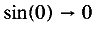).

When an unevaluated function application' is rendered there are a wide variety of appropriate renderings. The choice often depends on the function or operator being applied. Applications of basic operations such as plus are generally presented using an infix notation while applications of sin would use a more traditional functional notation such as sin (x). Consult the default rendering' for the operator being applied.

Applications of user-defined functions (see csymbol, fn) which are not evaluated by the receiving or rendering application would typically render using a traditional functional notation unless an alternative presentation is specified using the semantics tag.

#### 4.4.2.2.1. Discussion

The reln element was used in MathML 1.0 to construct an equation or relation. Relations were constructed in a manner exactly analogous to the use of apply. This usage is deprecated in MathML 2.0 in favour of the more generally usable apply.

The first child of reln is the relational operator, to be applied, with the other child elements as arguments.

See section 4.2.5 [Relations] for further details.

#### 4.4.2.2.2. Examples and Usage

<apply><eq/>
<ci> a </ci>
<ci> b </ci>
</apply>
<apply><lt/>
<ci> a </ci>
<ci> b </ci>
</apply>


1. a = b
2. a < b

#### 4.4.2.3.1. Discussion

The fn element makes explicit the fact that a more general (possibly constructed) MathML object is being used in the same manner as if it were a pre-defined function such as sin or plus.

In MathML1.0, fn was also the primary mechanism used to extend the collection of known' mathematical functions. This usage is now deprecated in favour of the more generally applicable csymbol element. (New functions may also be introduced by using declare in conjunction with a lambda expression.)

#### 4.4.2.3.2. Examples

1. <fn><ci> L </ci> </fn>
2. <apply>
<fn>
<apply>
<plus/>
<ci> f </ci>
<ci> g </ci>
</apply>
</fn>
<ci>z</ci>
</apply>


#### 4.4.2.3.3. Default Rendering

An fn object is rendered in the same way as its content. A rendering application may add additional adornments such as +parentheses to clarify the meaning.

1. L
2. (f + g)z

#### 4.4.2.4.1. Discussion

The interval element is used to represent simple mathematical intervals of the real number line. It takes an attribute closure which can take on any of the values open, closed, open-closed, or closed-open, with a default value of closed.

More general domains are constructed by using the condition and bvar elements to bind free variables to constraints.

The interval element expects either two child elements which evaluate to real numbers or one child element which is a condition defining the interval. interval accepts a closure attribute which specifies if the interval is open, closed, or half open.

#### 4.4.2.4.2. Examples

1. <interval>
<ci> a </ci>
<ci> b </ci>
</interval>
2. <interval closure="open-closed">
<ci> a </ci>
<ci> b </ci>
</interval>


1. [a, b]
2. (a, b]

#### 4.4.2.5.1. Discussion

The inverse element is applied to a function in order to construct a generic expression for the functional inverse of that function. (See also the discussion of inverse in section 4.4.2.5 [<inverse>]). As with other MathML functions, inverse may either be applied to arguments, or it may appear alone, in which case it represents an abstract inversion operator acting on other functions.

A typical use of the inverse element is in an HTML document discussing a number of alternative definitions for a particular function so that there is a need to write and define f(-1)(x).

To associate a particular definition with f(-1), use the definitionURL attribute.

#### 4.4.2.5.2. Examples

1. <apply><inverse/>
<ci> f </ci>
</apply>
2. <apply><inverse definitionURL="MyDefinition">
<ci> f </ci>
</apply>
3. <apply>
<apply><inverse/>
<ci type="matrix"> a </ci>
</apply>
<ci> A </ci>
</apply>


#### 4.4.2.5.3. Default Rendering

The default rendering for a functional inverse makes use of a parenthesized exponent as in f(-1)(x).

#### 4.4.2.6.1. Discussion

The sep element is to separate PCDATA into separate tokens for parsing the contents of the various specialized forms of the cn elements. For example sep is used when specifying the real and imaginary parts of a complex number (see section 4.4.1 [Token Elements]). If it occurs between MathML elements, it is a MathML error.

#### 4.4.2.6.2. Examples

<cn type="complex"> 3 <sep/> 4 </cn>


#### 4.4.2.6.3. Default Rendering

The sep element is not directly rendered. (see section 4.4.1 [Token Elements])

#### 4.4.2.7.1. Discussion

The condition element is used to place a condition on one or more free variables or identifiers. The conditions may be specified in terms of relations that are to be satisfied by the variables, including general relationships such as set membership.

It is used to define general sets and lists in situations where the elements cannot be explicitly enumerated. Condition contains either a single reln or apply element; the apply element is used to construct compound conditions. For example, it is used below to describe the set of all x such that x < 5. See the discussion on sets in section section 4.4.6 [Theory of Sets].

See section 4.2.6 [Conditions] for further details.

#### 4.4.2.7.2. Examples

1. <condition>
<apply><in/><ci> x </ci><ci type="set"> R </ci></apply>
</condition>
2. <condition>
<apply> <and/>
<apply><gt/><ci> x </ci><cn> 0 </cn></apply>
<apply><lt/><ci> x </ci><cn> 1 </cn></apply>
</apply>
</condition>
3. <apply>
<max/>
<bvar><ci> x </ci></bvar>
<condition>
<apply> <and/>
<apply><gt/><ci> x </ci><cn> 0 </cn></apply>
<apply><lt/><ci> x </ci><cn> 1 </cn></apply>
</apply>
</condition>
<apply>
<minus/>
<ci> x </ci>
<apply>
<sin/>
<ci> x </ci>
</apply>
</apply>
</apply>


#### 4.4.2.7.3. Default Rendering

1.2.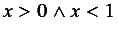3.#### 4.4.2.8.1. Discussion

The declare construct has two primary roles. The first is to change or set the default attribute values for a specific mathematical object. The second is to establish an association between a name' and an object. Once a declaration is in effect, the name' object acquires the new attribute settings, and (if the second object is present) all the properties of the associated object.

The various attributes of the declare element assign properties to the object being declared or determine where the declaration is in effect.

By default, the scope of a declaration is local' to the surrounding container element. Setting the value of the scope attribute to global extends the scope of the declaration to the enclosing math element. As discussed in section 4.3.2.8 [<scope>], MathML contains no provision for making document-wide declarations at present, though it is anticipated that this capability will be added in future revisions of MathML, when supporting technologies become available. declare takes one or two children. The first, mandatory, child is a ci containing the identifier being declared.

<declare type="vector"> <ci> V </ci> </declare>


The second, optional, child is a constructor initialising the variable

<declare type="vector">
<ci> V </ci>
<vector>
<cn> 1 </cn><cn> 2 </cn><cn> 3 </cn>
</vector>
</declare>


The constructor type and the type of the element declared must agree. For example, if the type attribute of the declaration is fn, the second child (constructor) must be an element equivalent to an fn element (This would include actual fn elements, lambda elements and any of the defined function in the basic set of content tags.) If no type is specified in the declaration then the type attribute of the declared name is set to the type of the constructor (second child) of the declaration. The type attribute of the declaration can be especially useful in the special case of the second element being a semantic tag.

#### 4.4.2.8.2. Attributes

All attributes are CDATA:

type
defines the MathML element type of the identifier declared.
scope
defines the scope of application of the declaration.
nargs
number of arguments for function declarations.
occurrence
describes operator usage as prefix, infix or function-model indications.
definitionURL
URL pointing to detailed semantics of the function.

#### 4.4.2.8.3. Examples

The declaration

<declare type="fn" nargs="2" scope="local">
<ci> f </ci>
<apply>
<plus/>
<ci> F </ci><ci> G </ci>
</apply>
</declare>


declares f to be a two-variable function with the property that f(x,y) = (F + G)(x,y).

The declaration

<declare type="fn">
<ci> J </ci>
<lambda>
<bvar><ci> x </ci></bvar>
<apply><ln/>
<ci> x </ci>
</apply>
</lambda>
</declare>


associates the name J with a one-variable function defined so that J(x) = ln y. (Note that because of the type attribute of the declare element, the second argument must be something of type fn, namely a known function like sin, an fn construct, or a lambda construct.)

The type attribute on the declaration is only necessary if if the type cannot be inferred from the type of the second argument.

Even when a declaration is in effect it is still possible to override attributes values selectively as in  <ci type="integer"> V </ci>. This capability is needed in order to write statements of the form Let S be a member of S'.

#### 4.4.2.8.4. Default Rendering

Since the declare construct is not directly rendered, most declarations are likely to be invisible to a reader. However, declarations can produce quite different effects in an application which evaluates or manipulates MathML content. While the declaration

<declare>
<ci> v </ci>
<vector>
<cn> 1 </cn>
<cn> 2 </cn>
<cn> 3 </cn>
</vector>
</declare>


is active the symbol v acquires all the properties of the vector, and even its dimension and components have meaningful values. This may affect how v is rendered by some applications, as well as how it is treated mathematically.

#### 4.4.2.9.1. Discussion

The lambda element is used to construct a user-defined function from an expression and one or more free variables. The lambda construct with n internal variables takes n+1 children. The first n children identify the variables which are used as placeholders in the last child for actual parameter values.

See section 4.2.2 [Containers] for further details.

#### 4.4.2.9.2. Examples

The following markup represents(x, sin x+1).

<lambda>
<bvar><ci> x </ci></bvar>
<apply><sin/>
<apply>
<plus/>
<ci> x </ci>
<cn> 1 </cn>
</apply>
</apply>
</lambda>


The following examples constructs a one argument function in which the argument b specifies the upper bound of a specific definite integral.

<lambda>
<bvar><ci> b </ci></bvar>
<apply>
<int/>
<bvar>
<ci> x </ci>
</bvar>
<lowlimit>
<ci> a </ci>
</lowlimit>
<uplimit>
<ci> b </ci>
</uplimit>
<apply><fn><ci> f </ci></fn>
<ci> x </ci>
</apply>
</apply>
</lambda>


Such constructs are often used conjunction with declare to construct new functions.

#### 4.4.2.9.3. Default Rendering(x, f(x)).

#### 4.4.2.10.1. Discussion

The <compose/> element represents the function composition operator. Note that MathML makes no assumption about the domain and range of the constituent functions in a composition; the domain of the resulting composition may be empty.

To override the default semantics for the <compose/> element, or to associate a more specific definition for function composition, use the definitionURL attribute.

See section 4.2.2 [Containers] for further details.

#### 4.4.2.10.2. Examples

The following markup represents.

<apply><compose/>
<fn><ci> f </ci></fn>
<fn><ci> g </ci></fn>
</apply>


The following markup represents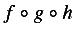.

<apply><compose/>
<ci type="fn"> f </ci>
<ci type="fn"> g </ci>
<ci type="fn"> h </ci>
</apply>


The following examples both represent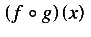.

<apply>
<apply><compose/>
<fn><ci> f </ci></fn>
<fn><ci> g </ci></fn>
</apply>
<ci> x </ci>
</apply>

<apply><fn><ci> f </ci></fn>
<apply>
<fn><ci> g </ci></fn>
<ci> x </ci>
</apply>
</apply>


#### 4.4.2.10.3. Default Rendering#### 4.4.2.11.1. Discussion

The ident element represents the identity function. MathML makes no assumption about the function space in which the identity function resides. That is, proper interpretation of the domain (and hence range) of the identity function depends on the context in which it is used.

To override the default semantics for the <ident/> element, or to associate a more specific definition, use the definitionURL attribute.

See section 4.2.2 [Containers] for further details.

#### 4.4.2.11.2. Examples

The following markup encoded the expression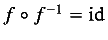.

<apply><eq/>
<apply><compose/>
<fn><ci> f </ci></fn>
<apply><inverse/>
<fn><ci> f </ci></fn>
</apply>
</apply>
<ident/>
</apply>


id

#### 4.4.3.1.1. Discussion

The quotient element is the operator used for division modulo a particular base. When the quotient operator is applied to integer arguments a and b, the result is the quotient of a divided by b'. That is, quotient returns the unique integer, q such that a = q b + r. (In common usage, q is called the quotient and r is the remainder.)

The quotient element takes the attribute definitionURL if it is desired to override the default semantics.

The quotient element is a binary arithmetic operator.

See section 4.2.3 [Functions, Operators and Qualifiers] for further details.

#### 4.4.3.1.2. Example

<apply><quotient/>
<ci> a </ci>
<ci> b </ci>
</apply>


Various mathematical applications will use this data in different ways. Editing applications might choose an image such as shown below, while a computationally based application would evaluate it to 2 when a=13 and b=5.

#### 4.4.3.1.3. Default Rendering

There is no commonly used notation for this concept. Some possible renderings are

1. quotient of a divided by b
2. integer part of a/b
3.#### 4.4.3.2.1. Discussion

The factorial element is used to construct factorials.

The factorial element takes the attribute definitionURL which may be used to override the default semantics.

The factorial element is a unary arithmetic operator.

See section 4.2.3 [Functions, Operators and Qualifiers] for further details.

#### 4.4.3.2.2. Example

<apply><factorial/>
<ci> n </ci>
</apply>


If this were evaluated at n = 5 it would evaluate to 120.

n!

#### 4.4.3.3.1. Discussion

The divide element is the division operator.

The divide element takes the attribute definitionURL which may be used to override the default semantics.

The divide element is a binary arithmetic operator. See section 4.2.3 [Functions, Operators and Qualifiers] for further details.

#### 4.4.3.3.2. Example

<apply>
<divide/>
<ci> a </ci>
<ci> b </ci>
</apply>


As a MathML expression, this does not evaluate. However, on receiving such an expression, some applications may attempt to evaluate and simplify the value. For example, when a=5 and b=2 some mathematical applications may evaluate this to 2.5 while others will treat is as a rational number.

a/b

#### 4.4.3.4.1. Discussion

The elements max and min are used to compare the values of their arguments. They return the maximum and minimum of these values respectively.

The max and min elements take the attribute definitionURL which can be used to override the default semantics.

The max and min elements are n-ary arithmetic operators. See section 4.2.3 [Functions, Operators and Qualifiers] for further details.

#### 4.4.3.4.2. Examples

When the objects are to be compared explicitly they are listed as arguments to the function as in:

<apply><max/>
<ci> a </ci>
<ci> b </ci>
</apply>


The elements to be compared may also be described using bound variables with a condition element and an expression to be maximised, as in:

<apply><min/>
<bvar><ci>x</ci></bvar>
<condition>
<apply><notin/><ci> x </ci><ci type="set"> B </ci></apply>
</condition>
<apply>
<power/>
<ci> x </ci>
<cn> 2 </cn>
</apply>
</apply>


Note that the bound variable may be implicit:

<apply><max/>
<condition>
<apply><and/>
<apply><in/><ci>x</ci><ci type="set">B</ci></apply>
<apply><notin/><ci>x</ci><ci type="set">C</ci></apply>
</apply>
</condition>
</apply>


#### 4.4.3.4.3. Default Rendering

1.2.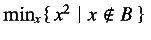3.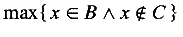#### 4.4.3.5.1. Discussion

The minus element is the subtraction operator.

The minus element takes the attribute definitionURL which can be used to override the default semantics.

The minus element can be used as a unary arithmetic operator (e.g. to represent -x) or as a binary arithmetic operator (e.g. to represent x-y). See section 4.2.3 [Functions, Operators and Qualifiers] for further details.

#### 4.4.3.5.2. Example

<apply> <minus/>
<ci> x </ci>
<ci> y </ci>
</apply>


If this were evaluated at x=5 and y=2 it would yield 3.

x - y

#### 4.4.3.6.1. Discussion

The plus element is the addition operator.

The plus element takes the attribute definitionURL which can be used to override the default semantics.

The plus element is an n-ary arithemtic operator. See section 4.2.3 [Functions, Operators and Qualifiers] for further details.

#### 4.4.3.6.2. Example

<apply>
<plus/>
<ci> x </ci>
<ci> y </ci>
<ci> z </ci>
</apply>


If this were evaluated at x = 5, y = 2 and z = 1 it would yield 8.

x + y + z

#### 4.4.3.7.1. Discussion

The power element is generic exponentiation operator. That is, when applied to arguments a and b, it returns the value the a to the power of b'.

The power element takes the attribute definitionURL which can be used to override the default semantics.

The power element is an binary arithmetic operator. See section 4.2.3 [Functions, Operators and Qualifiers] for further details.

#### 4.4.3.7.2. Example

<apply>
<power/>
<ci> x </ci>
<cn> 3 </cn>
</apply>


If this were evaluated at x= 5 it would yield 125.

x3

#### 4.4.3.8.1. Discussion

The rem element is the operator which returns the remainder' of a division modulo a particular base. When the rem operator is applied to integer arguments a and b, the result is the remainder of a divided by b'. That is, rem returns the unique integer, r such that a = q b + r, where r < q. (In common usage, q is called the quotient and r is the remainder.)

The rem element takes the attribute definitionURL which can be used to override the default semantics.

The rem element is a binary arithmetic operator. See section 4.2.3 [Functions, Operators and Qualifiers] for further details.

#### 4.4.3.8.2. Example

<apply><rem/>
<ci> a </ci>
<ci> b </ci>
</apply>


If this were evaluated at a = 15 and b = 8 it would yield 7.

a mod b

#### 4.4.3.9.1. Discussion

The times element is the multiplication operator.

times takes the attribute definitionURL which can be used to override the default semantics.

#### 4.4.3.9.2. Example

<apply> <times/>
<ci> a </ci>
<ci> b </ci>
</apply>


If this were evaluated at a = 5.5 and b = 3 it would yield 16.5.

a b

#### 4.4.3.10.1. Discussion

The root element is used to construct roots. The kind of root to be taken is specified by a degree element, which should be given as the first child of the apply element enclosing the root element. Thus, square roots correspond to the case where degree contains the value 2, cube roots correspond to 3, and so on. If no degree is present, a default value of 2 is used.

The root element takes the attribute definitionURL which can be used to override the default semantics.

The root element is an operator taking qualifiers. See section 4.2.4 [Operators taking Qualifiers] for further details.

#### 4.4.3.10.2. Example

The nth root of a is is given by

<apply> <root/>
<degree><ci> n </ci></degree>
<ci> a </ci>
</apply>


#### 4.4.3.10.3. Default Rendering#### 4.4.3.11.1. Discussion

The gcd element is used to denote the greatest common divisor of its arguments.

The gcd takes the attribute definitionURL which can be used to override the default semantics.

The gcd element is an n-ary operator. See section 4.2.3 [Functions, Operators and Qualifiers] for further details.

#### 4.4.3.11.2. Example

<apply> <gcd/>
<ci> a </ci>
<ci> b </ci>
<ci> c </ci>
</apply>


If this were evaluated at a = 15, b = 21, c = 48 it would yield 3.

gcd(a, b, c)

#### 4.4.3.12.1. Discussion

The and element is the boolean and' operator.

The and element takes the attribute definitionURL which can be used to override the default semantics.

The and element is an n-ary logical operator. See section 4.2.3 [Functions, Operators and Qualifiers] for further details.

#### 4.4.3.12.2. Example

<apply><and/>
<ci> a </ci>
<ci> b </ci>
</apply>


If this were evaluated and both a and b had truth values of true, then the value would be true.

#### 4.4.3.12.3. Default Rendering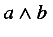#### 4.4.3.13.1. Discussion

The or element is the boolean or' operator.

The or element takes the attribute definitionURL which can be used to override the default semantics.

The or element is an n-ary logical operator. See section 4.2.3 [Functions, Operators and Qualifiers] for further details.

#### 4.4.3.13.2. Example

<apply><or/>
<ci> a </ci>
<ci> b </ci>
</apply>


#### 4.4.3.13.3. Default Rendering#### 4.4.3.14.1. Discussion

The xor element is the boolean exclusive or' operator.

xor takes the attribute definitionURL which can be used to override the default semantics.

The xor element is an n-ary logical operator. See section 4.2.3 [Functions, Operators and Qualifiers] for further details.

#### 4.4.3.14.2. Example

<apply><xor/>
<ci> a </ci>
<ci> b </ci>
</apply>


a xor b

#### 4.4.3.15. <not/>

The not operator is the boolean not' operator.

The not element takes the attribute definitionURL which can be used to override the default semantics.

The not element is an unary logical operator. See section 4.2.3 [Functions, Operators and Qualifiers] for further details.

#### 4.4.3.15.1. Example

<apply><not/>
<ci> a </ci>
</apply>


#### 4.4.3.15.2. Default Rendering#### 4.4.3.16.1. Discussion

The implies element is the boolean implies' relational operator.

The implies element takes the attribute definitionURL which can be used to override the default semantics.

The implies element is a binary logical operator. See section 4.2.5 [Relations] for further details.

#### 4.4.3.16.2. Example

<apply><implies/>
<ci> A </ci>
<ci> B </ci>
</apply>


Mathematical applications designed for the evaluation of such expressions would evaluate this to true when a = false and b = true.

#### 4.4.3.16.3. Default Rendering#### 4.4.3.17. <forall/>

The forall element represents the universal quantifier of logic. It must used in conjunction with one or more bound variables, an optional condition element, and an assertion, which may either take the form of an apply or reln element.

The forall element takes the attribute definitionURL which can be used to override the default semantics.

The forall element is an quantifier. See section 4.2.3 [Functions, Operators and Qualifiers] for further details.

#### 4.4.3.17.1. Examples

The first example encodes the sense of the expression for all x, x - x = 0'.

<apply>
<forall/>
<bvar><ci> x </ci></bvar>
<apply><eq/>
<apply>
<minus/><ci> x </ci><ci> x </ci>
</apply>
<cn>0</cn>
</apply>
</apply>


A more involved example, making use of an optional condition element encodes the sense of the expression: for all p, q insuch that p < q, p < q2.

<apply>
<forall/>
<bvar><ci> p </ci></bvar>
<bvar><ci> q </ci></bvar>
<condition>
<apply><and/>
<apply><in/><ci> p </ci><ci type="set"> Q </ci></apply>
<apply><in/><ci> q </ci><ci type="set"> Q </ci></apply>
<apply><lt/><ci> p </ci><ci> q </ci></apply>
</apply>
</condition>
<apply><lt/>
<ci> p </ci>
<apply>
<power/>
<ci> q </ci>
<cn> 2 </cn>
</apply>
</apply>
</apply>


A final example, utilizing both the forall and exists quantifiers, encodes the sense of the expression: for all n > 0, n in, there exist x, y, z insuch that xn + yn = zn.

<apply>
<forall/>
<bvar><ci> n </ci></bvar>
<condition>
<apply><and/>
<apply><gt/><ci> n </ci><cn> 0 </cn></apply>
<apply><in/><ci> n </ci><ci type="set"> Z </ci></apply>
</apply>
</condition>
<apply>
<exists/>
<bvar><ci> x </ci></bvar>
<bvar><ci> y </ci></bvar>
<bvar><ci> z </ci></bvar>
<condition>
<apply><and/>
<apply><in/><ci> x </ci><ci type="set"> Z </ci></apply>
<apply><in/><ci> y </ci><ci type="set"> Z </ci></apply>
<apply><in/><ci> z </ci><ci type="set"> Z </ci></apply>
</apply>
</condition>
<apply>
<eq/>
<apply>
<plus/>
<apply><power/><ci> x </ci><ci> n </ci></apply>
<apply><power/><ci> y </ci><ci> n </ci></apply>
</apply>
<apply><power/><ci> z </ci><ci> n </ci></apply>
</apply>
</apply>
</apply>


#### 4.4.3.17.2. Default Rendering

1. for all
2.#### 4.4.3.18. <exists/>

The exists element represents the existential quantifier of logic. It must used in conjuction with one or more bound variables, an optional condition element, and an assertion, which may either take the form of an apply or reln element.

The exists element takes the attribute definitionURL which can be used to override the default semantics.

The exists element is an quantifier. See section 4.2.3 [Functions, Operators and Qualifiers] for further details.

#### 4.4.3.18.1. Example

The following example encodes the sense of the expression there exists an x such that f(x) = 0'.

<apply>
<exists/>
<bvar><ci> x </ci></bvar>
<apply><eq/>
<apply>
<fn><ci> f </ci></fn>
<ci> x </ci>
</apply>
<cn>0</cn>
</apply>
</apply>


#### 4.4.3.18.2. Default Rendering

1. there exists
2.#### 4.4.3.19. <abs/>

The abs element represents the absolute value of a real quantity or the modulus of a complex quantity.

The abs element takes the attribute definitionURL which can be used to override the default semantics.

The abs element is a unary arithmetic operator. See section 4.2.3 [Functions, Operators and Qualifiers] for further details.

#### 4.4.3.19.1. Example

The following example encodes the absolute value of x.

<apply>
<abs/>
<ci> x </ci>
</apply>


|x|

#### 4.4.3.20. <conjugate/>

The conjugate element represents the complex conjugate of a complex quantity.

The conjugate element takes the attribute definitionURL which can be used to override the default semantics.

The conjugate element is a unary arithmetic operator. See section 4.2.3 [Functions, Operators and Qualifiers] for further details.

#### 4.4.3.20.1. Example

The following example encodes the conjugate of x + iy.

<apply><conjugate/>
<apply>
<plus/>
<ci> x </ci>
<apply><times/>
<cn> &ImaginaryI; </cn>
<ci> y </ci>
</apply>
</apply>
</apply>


#### 4.4.3.20.2. Default Rendering#### 4.4.4.1.1. Discussion

The eq element is the equals' relational operator.

The eq element takes the attribute definitionURL which can be used to override the default semantics.

The equals element is an n-ary relation. See section 4.2.5 [Relations] for further details.

#### 4.4.4.1.2. Example

<apply><eq/>
<ci> a </ci>
<ci> b </ci>
</apply>


If this were tested at a = 5.5 and b = 6 it would yield the truth value false'.

a = b

#### 4.4.4.2.1. Discussion

The neq element is the not equal to' relational operator.

neq takes the attribute definitionURL which can be used to override the default semantics.

The neq element is an binary relation. See section 4.2.5 [Relations] for further details.

#### 4.4.4.2.2. Example

<apply><neq/>
<ci> a </ci>
<ci> b </ci>
</apply>


If this were tested at a = 5.5 and b = 6 it would yield the truth value true'.

#### 4.4.4.2.3. Default Rendering#### 4.4.4.3.1. Discussion

The gt element is the greater than' relational operator.

The gt element takes the attribute definitionURL which can be used to override the default semantics.

The gt element is an n-ary relation. See section 4.2.5 [Relations] for further details.

#### 4.4.4.3.2. Example

<apply><gt/>
<ci> a </ci>
<ci> b </ci>
</apply>


If this were tested at a = 5.5 and b = 6 it would yield the truth value false'.

a > b

#### 4.4.4.4.1. Discussion

The lt element is the less than' relational operator.

The lt element takes the attribute definitionURL which can be used to override the default semantics.

The lt element is an n-ary relation. See section 4.2.5 [Relations] for further details.

#### 4.4.4.4.2. Example

<apply><lt/>
<ci> a </ci>
<ci> b </ci>
</apply>


If this were tested at a = 5.5 and b = 6 it would yield the truth value true'.

a < b

#### 4.4.4.5.1. Discussion

The geq element is the greater than or equal' relational operator.

The geq element takes the attribute definitionURL which can be used to override the default semantics.

The geq element is an n-ary relation. See section 4.2.5 [Relations] for further details.

#### 4.4.4.5.2. Example

<apply><geq/>
<ci> a </ci>
<ci> b </ci>
</apply>


If this were tested for a = 5.5 and b = 5.5 it would yield the truth value true'.

#### 4.4.4.5.3. Default Rendering#### 4.4.4.6.1. Discussion

The leq element is the less than or equal' relational operator.

The leq element takes the attribute definitionURL which can be used to override the default semantics.

The leq element is an n-ary relation. See section 4.2.5 [Relations] for further details.

#### 4.4.4.6.2. Example

<apply><leq/>
<ci> a </ci>
<ci> b </ci>
</apply>


If a = 5.4 and b = 5.5 this will yield the truth value true'.

#### 4.4.4.6.3. Default Rendering#### 4.4.5.1.1. Discussion

The int element is the operator element for an integral. The lower limit, upper limit and bound variable are given by (optional) child elements, lowlimit, uplimit and bvar in the enclosing apply element. The integrand is also specified as a child element of the enclosing apply element.

The domain of integration may alternatively be specified by using an interval element, or by a condition element. In such cases, if a bound variable of integration is intended, it must be specified explicitly. (The condition may involve more than one symbol.)

The int element takes the attribute definitionURL which can be used to override the default semantics.

The int element an operator taking qualifiers. See section 4.2.4 [Operators taking Qualifiers] for further details.

#### 4.4.5.1.2. Examples

This example specifies a lowlimit, uplimit, and bvar.

<apply>
<int/>
<bvar>
<ci> x </ci>
</bvar>
<lowlimit>
<cn> 0 </cn>
</lowlimit>
<uplimit>
<ci> a </ci>
</uplimit>
<apply>
<fn><ci> f </ci></fn>
<ci> x </ci>
</apply>
</apply>


This example specifies the domain of integration with an interval element.

<apply><int/>
<bvar>
<ci> x </ci>
</bvar>
<interval>
<ci> a </ci>
<ci> b </ci>
</interval>
<apply><cos/>
<ci> x </ci>
</apply>
</apply>


The final example specifies the domain of integration with an condition element.

<apply><int/>
<bvar>
<ci> x </ci>
</bvar>
<condition>
<apply><in/>
<ci> x </ci>
<ci type="set"> D </ci>
</apply>
</condition>
<apply><fn><ci> f </ci></fn>
<ci> x </ci>
</apply>
</apply>


#### 4.4.5.1.3. Default Rendering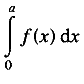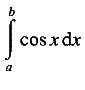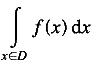#### 4.4.5.2.1. Discussion

The diff element is the differentiation operator element for functions of a single real variable. The bound variable is given by a bvar element which is a child of the containing apply element. The bvar elements may also contain a degree element, which specifies the order of the derivative to be taken.

The diff element takes the attribute definitionURL which can be used to override the default semantics.

The diff element is an operator taking qualifiers. See section 4.2.4 [Operators taking Qualifiers] for further details.

#### 4.4.5.2.2. Example

<apply><diff/>
<bvar>
<ci> x </ci>
</bvar>
<apply><fn><ci> f </ci></fn>
<ci> x </ci>
</apply>
</apply>


#### 4.4.5.2.3. Default Rendering#### 4.4.5.3.1. Discussion

The partialdiff element is the partial differentiation operator element for functions of several real variables. The bound variables are given by bvar elements, which are children of the containing apply element. The bvar elements may also contain a degree element, which specifies the order of the partial derivative to be taken in that variable.

The partialdiff element takes the attribute definitionURL which can be used to override the default semantics.

The partialdiff element is an operator taking qualifiers. See section 4.2.4 [Operators taking Qualifiers] for further details.

#### 4.4.5.3.2. Example

<apply>
<partialdiff/>
<bvar>
<ci> x </ci>
<degree>
<cn> 2 </cn>
</degree>
</bvar>
<bvar>
<ci> y </ci>
</bvar>
<apply><fn><ci> f </ci></fn>
<ci> x </ci>
<ci> y </ci>
</apply>
</apply>


#### 4.4.5.3.3. Default Rendering#### 4.4.5.4.1. Discussion

The lowlimit element is the container element used to indicate the lower limit' of an operator using qualifiers. For example, in an integral, it can be used to specify the lower limit of integration. Similarly, it is also used to specify the lower limit of an index for sums and products.

The meaning of the lowlimit element depends on the context it is being used in. For further details about how qualifiers are used in conjunction with operators taking qualifiers, consult section 4.2.4 [Operators taking Qualifiers].

#### 4.4.5.4.2. Example

<apply><int/>
<bvar>
<ci> x </ci>
</bvar>
<lowlimit>
<ci> a </ci>
</lowlimit>
<uplimit>
<ci> b </ci>
</uplimit>
<apply><fn><ci> f </ci></fn>
<ci> x </ci>
</apply>
</apply>


#### 4.4.5.4.3. Default Rendering

The default rendering of the lowlimit element and its contents depends on the context. In the preceding example, it should be rendered as a subscript to the integral sign:Consult the descriptions of individual operators which make use of the lowlimit construct for default renderings.

#### 4.4.5.5.1. Discussion

The uplimit element is the container element used to indicate the upper limit' of an operator using qualifiers. For example, in an integral, it can be used to specify the upper limit of integration. Similarly, it is also used to specify the upper limit of an index for sums and products.

The meaning of the uplimit element depends on the context it is being used in. For further details about how qualifiers are used in conjunction with operators taking qualifiers, consult section 4.2.4 [Operators taking Qualifiers].

#### 4.4.5.5.2. Example

<apply><int/>
<bvar>
<ci> x </ci>
</bvar>
<lowlimit>
<ci> a </ci>
</lowlimit>
<uplimit>
<ci> b </ci>
</uplimit>
<apply><fn><ci> f </ci></fn>
<ci> x </ci>
</apply>
</apply>


#### 4.4.5.5.3. Default Rendering

The default rendering of the uplimit element and its contents depends on the context. In the preceding example, it should be rendered as a superscript to the integral sign:Consult the descriptions of individual operators which make use of the uplimit construct for default renderings.

#### 4.4.5.6.1. Discussion

The bvar element is the container element for the bound variable' of an operation. For example, in an integral it specifies the variable of integration. In a derivative, it indicates which variable with respect to which a function is being differentiated. When the bvar element is used to quantifiy a derivative, the bvar element may contain a child degree element which specifies the order of the derivative with respect to that variable. The bvar element is also used for the internal variable in sums and products and for the bound variable used with the universal and existential quantifiers forall and exists.

The meaning of the bvar element depends on the context it is being used in. For further details about how qualifiers are used in conjunction with operators taking qualifiers, consult section 4.2.4 [Operators taking Qualifiers].

#### 4.4.5.6.2. Examples

<apply><diff/>
<bvar>
<ci> x </ci>
<degree>
<cn> 2 </cn>
</degree>
</bvar>
<apply>
<power/>
<ci> x </ci>
<cn> 2 </cn>
</apply>
</apply>

<apply><int/>
<bvar><ci> x </ci></bvar>
<condition>
<apply><in/><ci> x </ci><ci> D </ci></apply>
</condition>
<apply><fn><ci> f </ci></fn>
<ci> x </ci>
</apply>
</apply>


#### 4.4.5.6.3. Default Rendering

The default rendering of the bvar element and its contents depends on the context. In the preceding examples, it should be rendered as the x in the dx of the integral, and as the x in the denominator of the derivative symbol: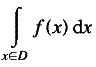Note that in the case of the derivative, the default rendering of the degree child of the bvar element is as an exponent.

Consult the descriptions of individual operators which make use of the uplimit construct for default renderings.

#### 4.4.5.7.1. Discussion

The degree element is the container element for the degree' or order' of an operation. There are a number basic mathematical constructs which come in families, such as derivatives and moments. Rather than introduce special elements for each of these families, MathML uses a single general construct, the degree element for this concept of order'.

The meaning of the degree element depends on the context it is being used in. For further details about how qualifiers are used in conjunction with operators taking qualifiers, consult section 4.2.4 [Operators taking Qualifiers].

#### 4.4.5.7.2. Example

<apply>
<partialdiff/>
<bvar>
<ci> x </ci>
<degree>
<ci> n </ci>
</degree>
</bvar>
<bvar>
<ci> y </ci>
<degree>
<ci> m </ci>
</degree>
</bvar>
<apply><sin/>
<apply> <times/>
<ci> x </ci>
<ci> y </ci>
</apply>
</apply>
</apply>


#### 4.4.5.7.3. Default Rendering

The default rendering of the degree element and its contents depends on the context. In the preceding example, the degree elements would be rendered as the exponents in the differentiation symbols:Consult the descriptions of individual operators which make use of the uplimit construct for default renderings.

#### 4.4.6.1.1. Discussion

The set element is the container element which constructs a set of elements. The elements of a set can be defined either by explicitly listing the elements, or by using the bvar and condition elements.

The set element is a constructor container. See section 4.2.2.2 [Constructors] for further details.

#### 4.4.6.1.2. Examples

<set>
<ci> b </ci>
<ci> a </ci>
<ci> c </ci>
</set>

<set>
<bvar><ci> x </ci></bvar>
<condition>
<apply><lt/>
<ci> x </ci>
<cn> 5 </cn>
</apply>
</condition>
</set>


1. {a, b, c}

2. {x | x < 5 }

#### 4.4.6.2.1. Discussion

The list element is the container element which constructs a list of elements. Elements can be defined either by explicitly listing the elements, or by using the bvar and condition elements.

Lists differ from sets in that there is an explicit order to the elements. Two orders are supported: lexicographic and numeric. The kind of ordering which should be used is specified by the order attribute.

The list element is a constructor container. See section 4.2.2.2 [Constructors] for further details.

#### 4.4.6.2.2. Examples

<list>
<ci> b </ci>
<ci> a </ci>
<ci> c </ci>
</list>

<list order="numeric">
<bvar><ci> x </ci></bvar>
<condition>
<apply><lt/>
<ci> x </ci>
<cn> 5 </cn>
</apply>
</condition>
</list>


1. [a, b, c]

2. [x | x < 5 ]

#### 4.4.6.3.1. Discussion

The union element is the operator for a set-theoretic union or join of two (or more) sets.

The union attribute takes the attribute definitionURL which can be used to override the default semantics.

The union element is an n-ary set operator. See section 4.2.3 [Functions, Operators and Qualifiers] for further details.

#### 4.4.6.3.2. Example

<apply><union/>
<ci type='set'> A </ci>
<ci type='set'> B </ci>
</apply>


#### 4.4.6.3.3. Default Rendering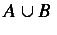#### 4.4.6.4.1. Discussion

The intersect element is the operator for the set-theoretic intersection or meet of two (or more) sets.

The intersect element takes the attribute definitionURL which can be used to override the default semantics.

The intersect element is an n-ary set operator. See section 4.2.3 [Functions, Operators and Qualifiers] for further details.

#### 4.4.6.4.2. Example

<apply><intersect/>
<ci type="set"> A </ci>
<ci type="set"> B </ci>
</apply>


#### 4.4.6.4.3. Default Rendering#### 4.4.6.5.1. Discussion

The in element is the relational operator used for a set-theoretic inclusion (is in' or is a member of').

The in element takes the attribute definitionURL which can be used to override the default semantics.

The in element is a binary set relation. See section 4.2.5 [Relations] for further details.

#### 4.4.6.5.2. Example

<apply>
<in/>
<ci> a </ci>
<ci type="set"> A </ci>
</apply>


#### 4.4.6.5.3. Default Rendering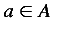#### 4.4.6.6.1. Discussion

The notin element is the relational operator element used for set-theoretic exclusion (is not in' or is not a member of').

The notin element takes the attribute definitionURL which can be used to override the default semantics.

The notin element is a binary set relation. See section 4.2.5 [Relations] for further details.

#### 4.4.6.6.2. Example

<apply><notin/>
<ci> a </ci>
<ci type='set'> A </ci>
</apply>


#### 4.4.6.6.3. Default Rendering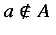#### 4.4.6.7.1. Discussion

The subset element is the relational operator element for a set-theoretic containment (is a subset of').

The subset element takes the attribute definitionURL which can be used to override the default semantics.

The subset element is an n-ary set relation. See section 4.2.5 [Relations] for further details.

#### 4.4.6.7.2. Example

<apply><subset/>
<ci type='set'> A </ci>
<ci type='set'> B </ci>
</apply>


#### 4.4.6.7.3. Default Rendering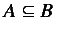#### 4.4.6.8.1. Discussion

The prsubset element is the relational operator element for set-theoretic proper containment (is a proper subset of').

The prsubset element takes the attribute definitionURL which can be used to override the default semantics.

The subset element is an n-ary set relation. See section 4.2.5 [Relations] for further details.

#### 4.4.6.8.2. Example

<apply>  <prsubset/>
<ci type='set'> A </ci>
<ci type='set'> B </ci>
</apply>


#### 4.4.6.8.3. Default Rendering#### 4.4.6.9.1. Discussion

The notsubset is the relational operator element for the set-theoretic relation is not a subset of'.

The notsubset element takes the attribute definitionURL which can be used to override the default semantics.

The notsubset element is a binary set relation. See section 4.2.5 [Relations] for further details.

#### 4.4.6.9.2. Example

<apply><notsubset/>
<ci type='set'> A </ci>
<ci type='set'> B </ci>
</apply>


#### 4.4.6.9.3. Default Rendering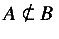#### 4.4.6.10.1. Discussion

The notprsubset is the operator element for the set-theoretic relation is not a proper subset of'.

The notprsubset takes the attribute definitionURL which can be used to override the default semantics.

The notprsubset element is a binary set relation. See section 4.2.5 [Relations] for further details.

#### 4.4.6.10.2. Example

<apply><notprsubset/>
<ci type='set'> A </ci>
<ci type='set'> B </ci>
</apply>


#### 4.4.6.10.3. Default Rendering#### 4.4.6.11.1. Discussion

The setdiff is the operator element for a set-theoretic difference of two sets.

The setdiff element takes the attribute definitionURL which can be used to override the default semantics. setdiff is a binary operator.

The setdiff element is a binary set operator. See section 4.2.3 [Functions, Operators and Qualifiers] for further details.

#### 4.4.6.11.2. Example

<apply><setdiff/>
<ci type='set'> A </ci>
<ci type='set'> B </ci>
</apply>


#### 4.4.6.11.3. Default Rendering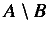#### 4.4.7.1.1. Discussion

The sum element denotes the summation operator. Upper and lower limits for the sum, and more generally a domains for the bound variables are specified using uplimit, lowlimit or a condition on the bound variables. The index for the summation is specified by a bvar element.

The sum element takes the attribute definitionURL which can be used to override the default semantics.

The sum element is an operator taking qualifiers. See section 4.2.4 [Operators taking Qualifiers] for further details.

#### 4.4.7.1.2. Examples

<apply><sum/>
<bvar>
<ci> x </ci>
</bvar>
<lowlimit>
<ci> a </ci>
</lowlimit>
<uplimit>
<ci> b </ci>
</uplimit>
<apply><fn><ci> f </ci></fn>
<ci> x </ci>
</apply>
</apply>

<apply><sum/>
<bvar>
<ci> x </ci>
</bvar>
<condition>
<apply> <in/>
<ci> x </ci>
<ci type="set"> B </ci>
</apply>
</condition>
<apply><fn><ci> f </ci></fn>
<ci> x </ci>
</apply>
</apply>


#### 4.4.7.1.3. Default Rendering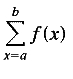#### 4.4.7.2.1. Discussion

The product element denotes the product operator. Upper and lower limits for the product, and more generally a domains for the bound variables are specified using uplimit, lowlimit or a condition on the bound variables. The index for the product is specified by a bvar element.

The product element takes the attribute definitionURL which can be used to override the default semantics.

The product element is an operator taking qualifiers. See section 4.2.4 [Operators taking Qualifiers] for further details.

#### 4.4.7.2.2. Examples

<apply>
<product/>
<bvar>
<ci> x </ci>
</bvar>
<lowlimit>
<ci> a </ci>
</lowlimit>
<uplimit>
<ci> b </ci>
</uplimit>
<apply><fn><ci> f </ci></fn>
<ci> x </ci>
</apply>
</apply>

<apply>
<product/>
<bvar>
<ci> x </ci>
</bvar>
<condition>
<apply> <in/>
<ci> x </ci>
<ci type="set"> B </ci>
</apply>
</condition>
<apply><fn><ci> f </ci></fn>
<ci> x </ci>
</apply>
</apply>


See section 4.2 [Content Element Usage Guide] for further details.

#### 4.4.7.2.3. Default Rendering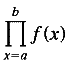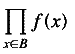#### 4.4.7.3.1. Discussion

The limit element is operation of taking a limit of a sequence. The limit point is expressed by specifying a lowlimit and a bvar or by specifying a condition on one or more bound variables.

The limit element takes the attribute definitionURL which can be used to override the default semantics.

The limit element is an operator taking qualifiers. See section 4.2.4 [Operators taking Qualifiers] for further details.

#### 4.4.7.3.2. Examples

<apply><limit/>
<bvar>
<ci> x </ci>
</bvar>
<lowlimit>
<cn> 0 </cn>
</lowlimit>
<apply><sin/>
<ci> x </ci>
</apply>
</apply>

<apply><limit/>
<bvar>
<ci> x </ci>
</bvar>
<condition>
<apply>
<tendsto type="above">
<ci> x </ci>
<ci> a </ci>
</apply>
</condition>
<apply><sin/>
<ci> x </ci>
</apply>
</apply>


#### 4.4.7.3.3. Default Rendering#### 4.4.7.4.1. Discussion

The tendsto element is used to express the relation that a quantity is tending to a specified value.

The tendsto element takes the attributes type to set the direction from which the the limiting value is approached and definitionURL which can be used to override the default semantics.

The tendsto element is a binary relational operator. See section 4.2.5 [Relations] for further details.

#### 4.4.7.4.2. Examples

<apply>
<tendsto type="above">
<apply>
<power/>
<ci> x </ci>
<cn> 2 </cn>
</apply>
<apply>
<power/>
<ci> a </ci>
<cn> 2 </cn>
</apply>
</apply>


To express, one might use vectors, as in:

<apply>
<tendsto/>
<vector>
<ci> x </ci>
<ci> y </ci>
</vector>
<vector>
<apply><fn><ci> f </ci></fn>
<ci> x </ci>
<ci> y </ci>
</apply>
<apply><fn><ci> g </ci></fn>
<ci> x </ci>
<ci> y </ci>
</apply>
</vector>
</apply>


#### 4.4.7.4.3. Default Rendering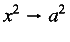#### 4.4.8. Elementary classical functions

The names of the common trigonometric functions supported by MathML are listed below. Since their standard interpretations are widely known, they are discussed as a group.

 sin cos tan sec csc cot sinh cosh tanh sech csch coth arcsin arccos arctan

#### 4.4.8.1. Discussion

These operator elements denote the standard trigonometrical functions.

These elements all take the attribute definitionURL, which can be used to override the default semantics.

They are all unary trigonometric operators. See section 4.2.3 [Functions, Operators and Qualifiers] for further details.

#### 4.4.8.2. Examples

<apply><sin/>
<ci> x </ci>
</apply>

<apply><sin/>
<apply>
<plus/>
<apply><cos/>
<ci> x </ci>
</apply>
<apply>
<power/>
<ci> x </ci>
<cn> 3 </cn>
</apply>
</apply>
</apply>


sin x

sin(cos x + x3)

#### 4.4.8.4.1. Discussion

The exp element represents the exponential function associated with the inverse of the ln function. In particular, exp(1) is approximately 2.718281828.

exp takes the attribute definitionURL which may be used to override the default semantics.

The exp element is a unary arithmetic operator. See section 4.2.3 [Functions, Operators and Qualifiers] for further details.

#### 4.4.8.4.2. Example

<apply><exp/>
<ci> x </ci>
</apply>


#### 4.4.8.4.3. Default Rendering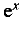#### 4.4.8.5.1. Discussion

The ln element is the natural logarithm operator.

The ln element takes the attribute definitionURL which can be used to override the default semantics.

The ln element is an unary calculus operator. See section 4.2.3 [Functions, Operators and Qualifiers] for further details.

#### 4.4.8.5.2. Example

<apply><ln/>
<ci> a </ci>
</apply>


If a = e this will yield the value 1.

ln a

#### 4.4.8.6.1. Discussion

The log element is the operator which returns a logarithm to a given base. The base may be specified using a logbase element, which should be the first child of the containing apply element. If the logbase element is not present, a default base of 10 is assumed.

The log element takes the attribute definitionURL which can be used to override the default semantics.

The log element can be used as either an operator taking qualifiers or a unary calculus operator. See section 4.2.3 [Functions, Operators and Qualifiers] for further details.

#### 4.4.8.6.2. Example

<apply> <log/>
<logbase>
<cn> 3 </cn>
</logbase>
<ci> x </ci>
</apply>


This markup represents the base 3 log of x'. For natural logarithms base e, the ln element should be used instead.

log3 x

#### 4.4.9.1.1. Discussion

mean is the operator element for a mean or average.

mean takes the attribute definitionURL, which can be used to override the default semantics.

#### 4.4.9.1.2. Example

mean is an n-ary operator.

<apply><mean/>
<ci> X </ci>
</apply>


See section 4.2 [Content Element Usage Guide] for further details.

#### 4.4.9.1.3. Default Rendering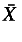or#### 4.4.9.2.1. Discussion

sdev is the operator element for the standard deviation.

sdev takes the attribute definitionURL, which can be used to override the default semantics.

#### 4.4.9.2.2. Example

sdev is an n-ary operator.

<apply><sdev/>
<ci> X </ci>
</apply>


See section 4.2 [Content Element Usage Guide] for further details.

#### 4.4.9.2.3. Default Rendering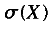#### 4.4.9.3.1. Discussion

variance is the operator element for the statistical variance.

variance takes the attribute definitionURL, which can be used to override the default semantics.

#### 4.4.9.3.2. Example

variance is an n-ary operator.

<apply><variance/>
<ci> X </ci>
</apply>


See section 4.2 [Content Element Usage Guide] for further details.

#### 4.4.9.3.3. Default Rendering#### 4.4.9.4.1. Discussion

median is the operator element for the median .

median takes the attribute definitionURL, which can be used to override the default semantics.

#### 4.4.9.4.2. Example

median is an n-ary operator.

<apply><median/>
<ci> X </ci>
</apply>


See section 4.2 [Content Element Usage Guide] for further details.

median(X)

#### 4.4.9.5.1. Discussion

mode is the operator for the statistical mode.

mode takes the attribute definitionURL, which can be used to override the default semantics.

#### 4.4.9.5.2. Example

mode is an n-ary operator.

<apply>
<mode/>
<ci> X </ci>
</apply>


See section 4.2 [Content Element Usage Guide] for further details.

mode(X)

#### 4.4.9.6.1. Discussion

The moment element represents statistical moments. Use degree for the n in n-th moment'.

moment takes the attribute definitionURL, which can be used to override the default semantics.

#### 4.4.9.6.2. Example

moment is an operator taking qualifiers.

<apply><moment/>
<degree>
<cn> 3 </cn>
</degree>
<ci> X </ci>
</apply>


See section 4.2 [Content Element Usage Guide] for further details.

#### 4.4.9.6.3. Default Rendering#### 4.4.10.1.1. Discussion

vector is the container element for a vector. The child elements form the components of the vector.

For purposes of interaction with matrices and matrix multiplication vectors are regarded as equivalent to a matrix consisting of a single column and the transpose of a vector behaves the same as a matrix consisting of a single row.

#### 4.4.10.1.2. Example

vector is a constructor element.

<vector>
<cn> 1 </cn>
<cn> 2 </cn>
<cn> 3 </cn>
<ci> x </ci>
</vector>


See section 4.2 [Content Element Usage Guide] for further details.

(1, 2, 3, x)

#### 4.4.10.2.1. Discussion

The matrix is the container element for matrixrow's. matrixrow's contain the elements of a matrix.

#### 4.4.10.2.2. Example

matrix is a constructor element.

<matrix>
<matrixrow>
<cn> 0 </cn> <cn> 1 </cn> <cn> 0 </cn>
</matrixrow>
<matrixrow>
<cn> 0 </cn> <cn> 0 </cn> <cn> 1 </cn>
</matrixrow>
<matrixrow>
<cn> 1 </cn> <cn> 0 </cn> <cn> 0 </cn>
</matrixrow>
</matrix>


See section 4.2 [Content Element Usage Guide] for further details.

#### 4.4.10.2.3. Default Rendering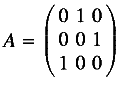#### 4.4.10.3.1. Discussion

The matrixrow element is the container element for the rows of a matrix.

#### 4.4.10.3.2. Example

matrixrow is a constructor element.

<matrixrow>
<cn> 1 </cn>
<cn> 2 </cn>
</matrixrow>
<matrixrow>
<cn> 3 </cn>
<ci> x </ci>
</matrixrow>


See section 4.2 [Content Element Usage Guide] for further details.

#### 4.4.10.3.3. Default Rendering

Matrix rows are not directly rendered by themselves outside of the context of a matrix.

#### 4.4.10.4.1. Discussion

The determinant element is the operator for constructing the determinant of a matrix.

determinant takes the attribute definitionURL, which can be used to override the default semantics.

#### 4.4.10.4.2. Example

determinant is a unary operator.

<apply><determinant/>
<ci type="matrix"> A </ci>
</apply>


See section 4.2 [Content Element Usage Guide] for further details.

det A

#### 4.4.10.5.1. Discussion

The transpose element is the operator for constructing the transpose of a matrix.

transpose takes the attribute definitionURL, which can be used to override the default semantics.

#### 4.4.10.5.2. Example

transpose is a unary operator.

<apply><transpose/>
<ci type="matrix"> A </ci>
</apply>


See section 4.2 [Content Element Usage Guide] for further details.

At

#### 4.4.10.6.1. Discussion

The selector element is the operator for indexing into vectors matrices and lists. It accepts one or more arguments. The first argument identifies the vector, matrix or list from which the selection is taking place, and the second and subsequent arguments, if any, indicate the kind of selection taking place.

When selector is used with a single argument, it should be interpreted as giving the sequence of all elements in the list, vector or matrix given. The ordering of elements in the sequence for a matrix is understood to be first by column, then by row. That is, for a matrix (ai,j), where the indices denote row and column, the ordering would be a1,1, a1,2, ... a2,1, a2,2 ... etcetera.

When three arguments are given, the last one is ignored for a list or vector, and in the case of a matrix, the second and third arguments specify the row and column of the selected element.

When two arguments are given, and the first is a vector or list, the second argument specifies an element in the list or vector. When a matrix and only one index i is specified as in

<apply><selector/>
<matrix>
<matrixrow>
<cn> 1 </cn> <cn> 2 </cn>
</matrixrow>
<matrixrow>
<cn> 3 </cn> <cn> 4 </cn>
</matrixrow>
</matrix>
<cn> 1 </cn>
</apply>


it refers to i-th matrixrow. Thus, the preceding example selects the following row:

<matrixrow> <cn> 1 </cn> <cn> 2 </cn> </matrixrow>


selector takes the attribute definitionURL, which can be used to override the default semantics.

selector is classified as an n-ary linear algebra operator even though it can take only one, two, or three arguments.

#### 4.4.10.6.2. Example

<apply><selector/>
<ci type="matrix"> A </ci>
<cn> 3 </cn>
<cn> 2 </cn>
</apply>


#### 4.4.10.6.3. Default Rendering

The selector construct renders the same as the expression which is selects.

#### 4.4.11. Semantic Mapping Elements

The use of the semantic mapping elements is explained in section 4.2.7 [Syntax and Semantics].

#### 4.4.11.1. Discussion

The annotation element is the container element for a semantic annotation in a non-XML format.

annotation takes the attribute encoding to define the encoding being used.

#### 4.4.11.2. Example

annotation is a semantic mapping element. It is always used with semantics.

<semantics>
<apply>
<plus/>
<apply><sin/>
<ci> x </ci>
</apply>
<cn> 5 </cn>
</apply>
<annotation encoding="TeX">
\sin x + 5
</annotation>
</semantics>


#### 4.4.11.3. Default Rendering

None. The information contained in annotations may optionally be used by a renderer able to process the kind of annotation given.

#### 4.4.11.4.1. Discussion

The semantics element is the container element which associates additional representations with a given MathML construct. The semantics element has as its first child the expression being annotated, and the subsequent children are the annotations. There is no restriction on the kind of annotation that can be attached using the semantics element. For example, one might give a TEX encoding, or computer algebra input in an annotation.

The representations which are XML based are enclosed in an annotation-xml element while those representations which are to be parsed as PCDATA are enclosed in an annotation element.

semantics takes the attribute definitionURL which can be used to reference an external source for some or all of the semantic information.

An important purpose of the semantics construct is to associate specific semantics with a particular presentation, or additional presentation information with a content construct. The default rendering of a semantics element is the default rendering of its first child. When a MathML-presentation annotation is provided, a MathML renderer may optionally use this information to render the MathML construct. This would typically be the case when the first child is a MathML content construct and the annotation is provided to give a preferred rendering differing from the default for the content elements.

#### 4.4.11.4.2. Examples and Usage

semantics is a semantic mapping element.

<semantics>
<apply>
<plus/>
<sin/>
<ci> x <ci/>
</apply>
<ame="cn"/> 5 </cn>
</apply>
<annotation encoding="Maple">
sin(x) + 5
</annotation>
<annotation-xml encoding="MathML-Presentation">
...
...
</annotation-xml>
<annotation encoding="Mathematica">
Sin[x] + 5
</annotation>
<annotation encoding="TeX">
\sin x + 5
</annotation>
<annotation-xml encoding="OpenMath">
<OMA>..</OMA>
</annotation-xml>
</semantics>


#### 4.4.11.4.3. Default Rendering

The default rendering of a semantics element is the default rendering of its first child.

#### 4.4.11.5.1. Discussion

The annotation-xml container element is used to contain representations that are XML based. It is always used together with the semantics element, and takes the attribute encoding to define the encoding being used.

annotation-xml is a semantic mapping element.

#### 4.4.11.5.2. Example

<semantics>
<apply>
<plus/>
<apply><sin/>
<ci> x </ci>
</apply>
<cn> 5 </cn>
</apply>
<annotation-xml encoding="OpenMath">
<OMA><OMS name="plus">
<OMA><OMS name="sin">
<OMV name="x">
</OMA>
5
</OMA>
</annotation-xml>
</semantics>


See also semantics in the Usage Guide.

#### 4.4.11.5.3. Default Rendering

None. The information may optionally be used by a renderer able to process the kind of annotation given.